Vector Thrust

timesymmetry | Iceberg Interactive | Released 2012

Fast pace cellshading flying action. Soar the skies as you take on the most vicious enemies above the ground. Experience an airborne adventure in an all new art style that will take you to something different and exciting. With the possibility to add just about anything you can imagine into the game, the sky it's not the limit. It's just the beginning.

Tutorial 002: Basic Map Creation

The first steps how to add a new original map to the game.

Posted by on - Basic Mapping/Technical

This tutorial will be part of the series of tutorials that will encompass the whole steps in creation and exporting to the game.
This in particular will focus only in making a basic map as simple as possible and adding it to the game.

First the template for the map file. (in versions 0.197 and onwards the template file will be include in the game folder)

String code:
MapFile = "String"

[scene]
AmbientLight = "Vector3"

TypeBG = "uint"
//TypeBG:
//-0 Not Used
//-1 skyX
//-2 skybox
//-3 skydome

BGmaterial = "String"

NoFog = "bool"
FogStart = "Real"

NoSun = "bool"
Sun_AutoConfig = "bool" (unavailable for now)
Sun_AutoConfig_Hour = "Vector2"

Sun_position = "Vector3" (only for TypeBG !=0)
Sun_DiffuseColour = "Vector3" (only for TypeBG !=0)
Sun_SpecularColour= "Vector3" (only for TypeBG !=0)

Sun_LensFlare = "String"

NoGroundLightScater  = "bool"
ScaterLight_DiffuseColour = "Vector3"
ScaterLight_SpecularColour= "Vector3"

nDirectLights = "uint"
nSNodes = "uint"
nCollMesh= "uint"
StaticGeom="uint"

//-------------------------------------------------------------------------
//-------------------------------CLOUDS------------------------------------

[Clouds]
numLayers=uint

//TypeBG:
//-0 Not Used
//-1 Dome
//-2 Cylinder
//-3 Plane
//-4 Bilboard

//Type 1:
Clayer_#_type=1
Clayer_#_Ypos= "Real"
Clayer_#_StartFadePerc= "Real" (starts fading as the camera aproaches the Ypos, for example StartFadePerc=0.8 and Ypos=4000)
Clayer_#_Texture= "String"
Clayer_#_ModelType="uint"
//ModelType:
//-0 Dome
//-1 Curved plane

//Type 2:
Clayer_#_type=2
Clayer_#_Ypos="Real"
Clayer_#_Texture= "String"
Clayer_#_ModelType="uint"
//ModelType:
//-0 Cylinder

//Type 3:
Clayer_#_type=3
Clayer_#_Ypos="Real"
Clayer_#_Texture= "String"
Clayer_#_ModelType="uint"
//ModelType:
//-0 Plane F
//-1 Plane L

//Type 4:
Clayer_#_type=4
Clayer_#_Ypos="Real"
Clayer_#_YvarRange="Real"
Clayer_#_Texture="String"
Clayer_#_NumClouds="uint"
Clayer_#_BillDefDimen = "Vector2"
Clayer_#_BillType = "uint"
//BillType:
//-0 omni direction
//-1 Vertical billboard
//-2 horizontal billboard

//-------------------------------------------------------------------------
//--------------------------------TREES------------------------------------

[Trees]
NumLayers = "uint"

Layer_#_Dispersion= "uint" (in a 20 km^2 area, max 50 000)
Layer_#_HighTexture= "String"
Layer_#_MedTexture= "String"
Layer_#_LowTexture= "String"
Layer_#_Size= "Vector2"
Layer_#_FullMap= "bool" (if full map, there is no need to define bounding zone)
Layer_#_BoundingZone= "Vector4" (x Min x Max z Min z Max)
Layer_#_VerticalBoundingZ= "Vector2"
Layer_#_NoSnapGround= "bool"
Layer_#_NoTerrRestrict= "bool"
Layer_#_VisibleRange= "Vector2" (losest range fardest range)
Layer_#_NumManualPoints =  "uint"
Layer_#_ManualPoint_#_Pos=  "Vector3"

//-------------------------------------------------------------------------
//-----------------------------COLISIONS-----------------------------------

[Colisions]
Col_#_Mesh= "String"
Col_#_Pos="Vector3"
Col_#_TreeL_#_NoTrees = "bool"
Col_#_TreeL_#_Prob="Real" [0.0 to 1.0]
Col_#_ColType="uint"
//ColType:
//-0 Basic
//-1 Dirt
//-2 Mud
//-3 Grass
//-4 Concrete
//-5 Paved
//-6 Snow
//-7 Ice
//-8 Rock
//-9 Sand
//-10 Deep Water
//-11 Shallow Water
//-12 Skin
//-13 Armored Skin
//-14 Wood
//-15 Structure
//-16 Reinforced Structure
//-17 Non Armored Vehicle
//-18 Light Armored Vehicle
//-19 Heavy Armored Vehicle
//-20 Reactive Armor

//-------------------------------------------------------------------------
//---------------------------SCENE NODES-----------------------------------

[sn_#]
sn_name="String"
sn_IsSubNode = "bool"
sn_ParentSn_name = "String"

usa_eixos_3dMax="bool" (version 0.196 or below)
use_3dMax_Axis="bool" (only for version 0.197 or above)
//with this option on the maker can copy paste the coordonates without having to worry about the convertions
//how to convert 3dmax units: [X Y Z] -> in the game are [X Z -Y]

sn_Pos="Vector3"
sn_Yaw="Real"
sn_Pitch="Real"
sn_Roll="Real"
sn_Scale="Vector3"

sn_nMeshes= "uint"
sn_nReacts= "uint"

snMesh_Me_# = "String" (mesh file)
snMesh_Vis_# = "bool" (visible)
snMesh_UseCustomMat_# = "bool"
snMesh_CustomMatName_# = "String"
snMesh_AnimNa_# = "String" (Animation name)
snMesh_AnimSpd_# = "Real"

snReact_Name_#  = "String" (Reactor file)

//-------------------------------------------------------------------------
//-----------------------STATIC GEOMETRY-----------------------------------

[static_#]
Static_name="String"

usa_eixos_3dMax="bool" (version 0.196 or below)
use_3dMax_Axis="bool" (only for version 0.197 or above)
//with this option on the maker can copy paste the coordonates without having to worry about the convertions
//how to convert 3dmax units: [X Y Z] -> in the game are [X Z -Y]

Static_Origin="Vector3"
Static_RegionDimensions="Vector3"
Static_setRenderingDistance="Real"
Static_Visible="bool"
Static_Mesh= "String"
Static_Mesh_Name="String" (only for version 0.196 or below)
Static_nMeshes= "uint"

stMesh_pos_# = "Vector3"
stMesh_yaw_# = "Real"
stMesh_scale_# = "Vector3"

//-------------------------------------------------------------------------
//-------------------------SCENE OBJECTS-----------------------------------

[SceneObjects]
numObjects="uint"

SceneO_#_CallN = "String"
SceneO_#_Pos = "Vector3"
SceneO_#_Yaw = "Real"

//-------------------------------------------------------------------------
//------------------------TERRAIN MATERIALS--------------------------------

[TerrainMaterials]
NumMaterials = "uint"
DetailTexture = "String"
WaterTexture = "String"
OceanMatName = "String"
WaterToneOverlay = "String"
TerrainToneOverlay= "String"

Mat_#_MaterialName="String"
Mat_#_TextureName="String"
Mat_#_HasWater = "bool"
Mat_#_IsTile = "bool"

Now how to make a map, for this tutorial it was used:
3d max 9
Ogre easy exporter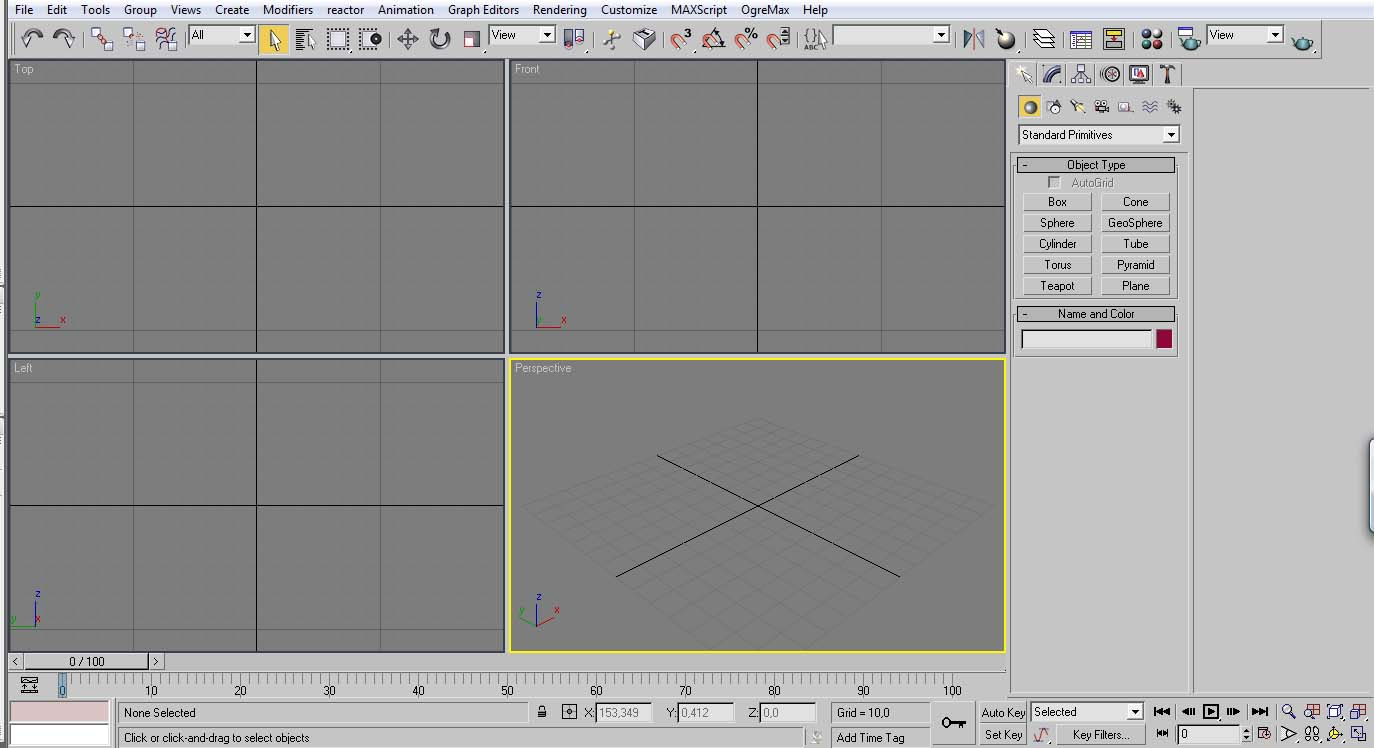Start by open a clean project of the 3d max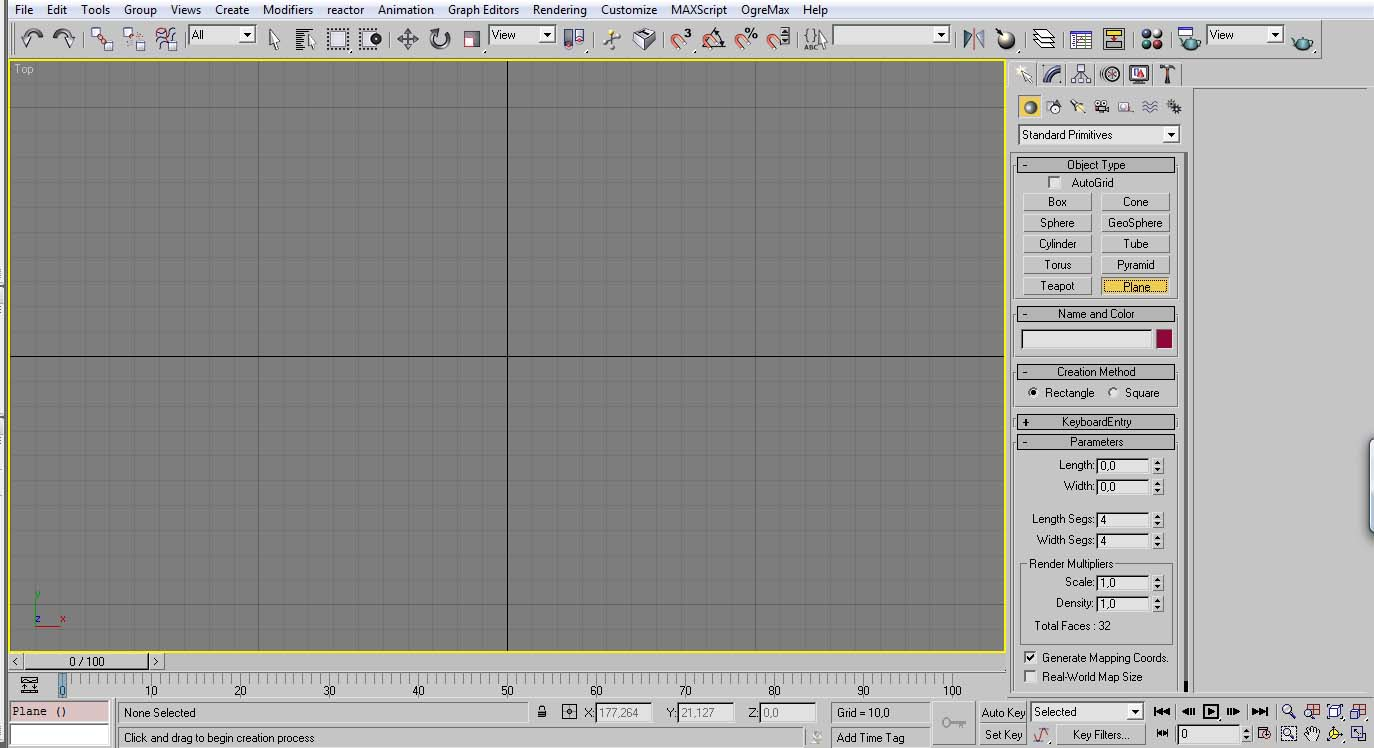Select the plane button.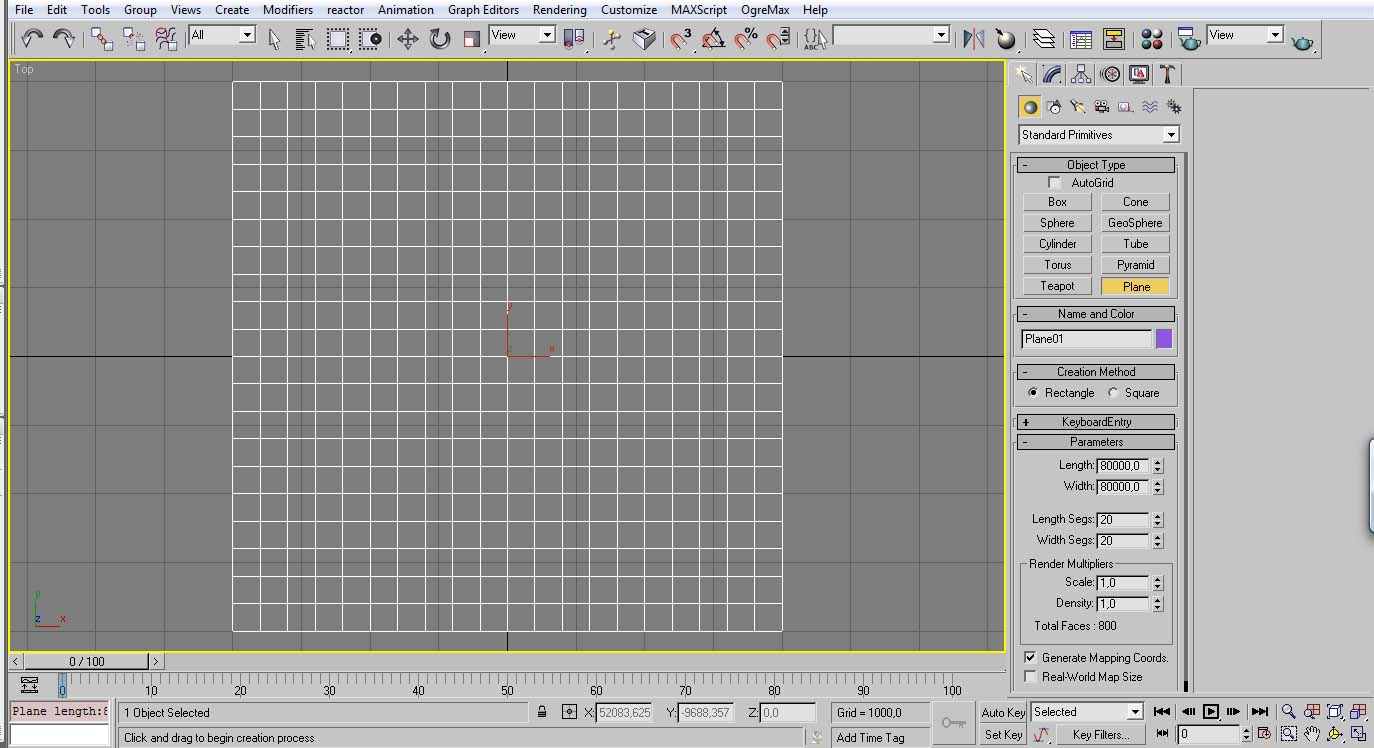Create a plane.
The size of the map depends what the maker want to do with it.
Currently the official maps have 80km x 80km in size. Be the way, while there isn’t any size restriction in the maps always consider how much time it is required to go to one end to another.
Another thing that should be considered is if using the present tileset in the game they were made for 4kmx4km squares in mind.
So set the appropriate sub division for the size you use.
In the 80km size it is required 20 sub divisions.
“80/4=20”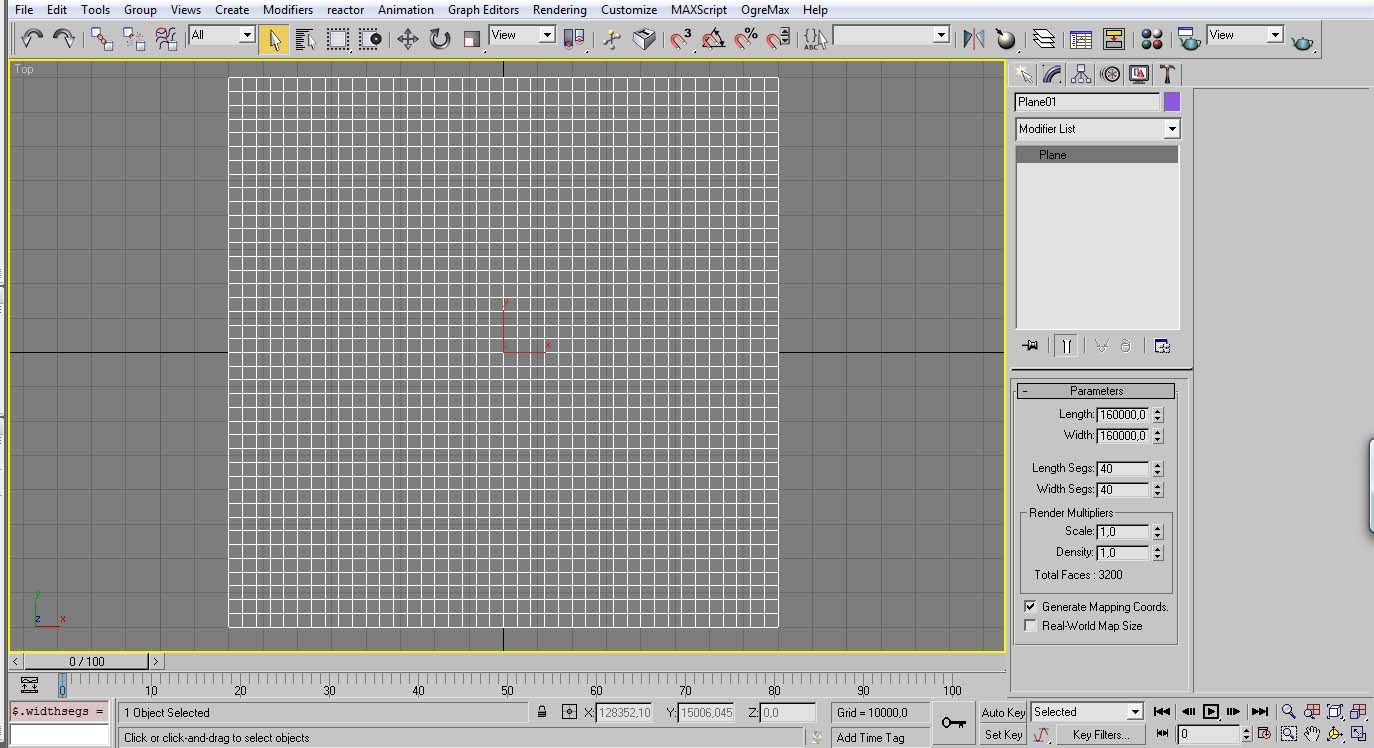After deciding the size of our playground, we must then set an additional extension in size.
We don’t what to see the map to end abruptly, so at lest we need to add more terrain to cover the player draw distance.
In the game, the currently maximum draw distance is 35 km, but just to be safe it is better to add at least 40km to every side.
So in a 80km size map, it becomes a 160 km size. (don’t forget to adjust the sub divisions!)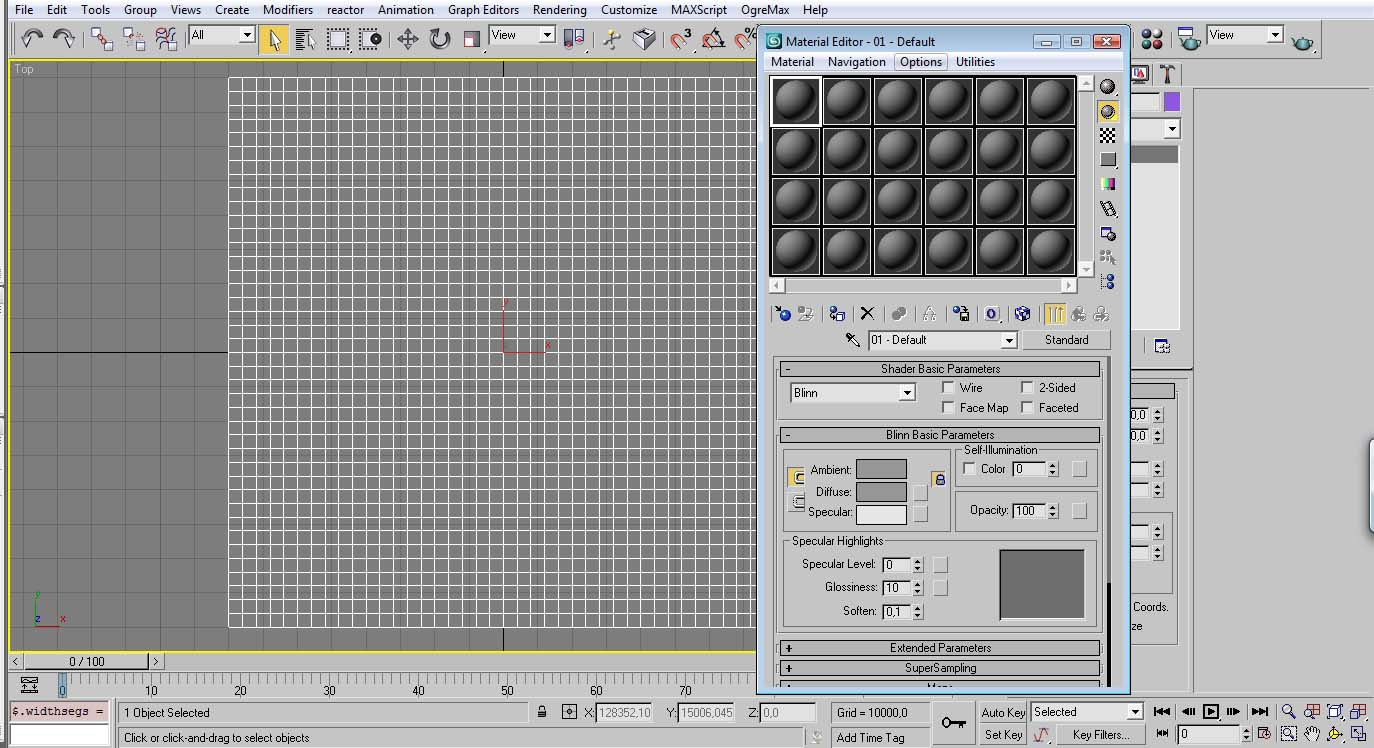The next step is to adjust the materials to load the tiles we may require for the map.
Press M to open the material editor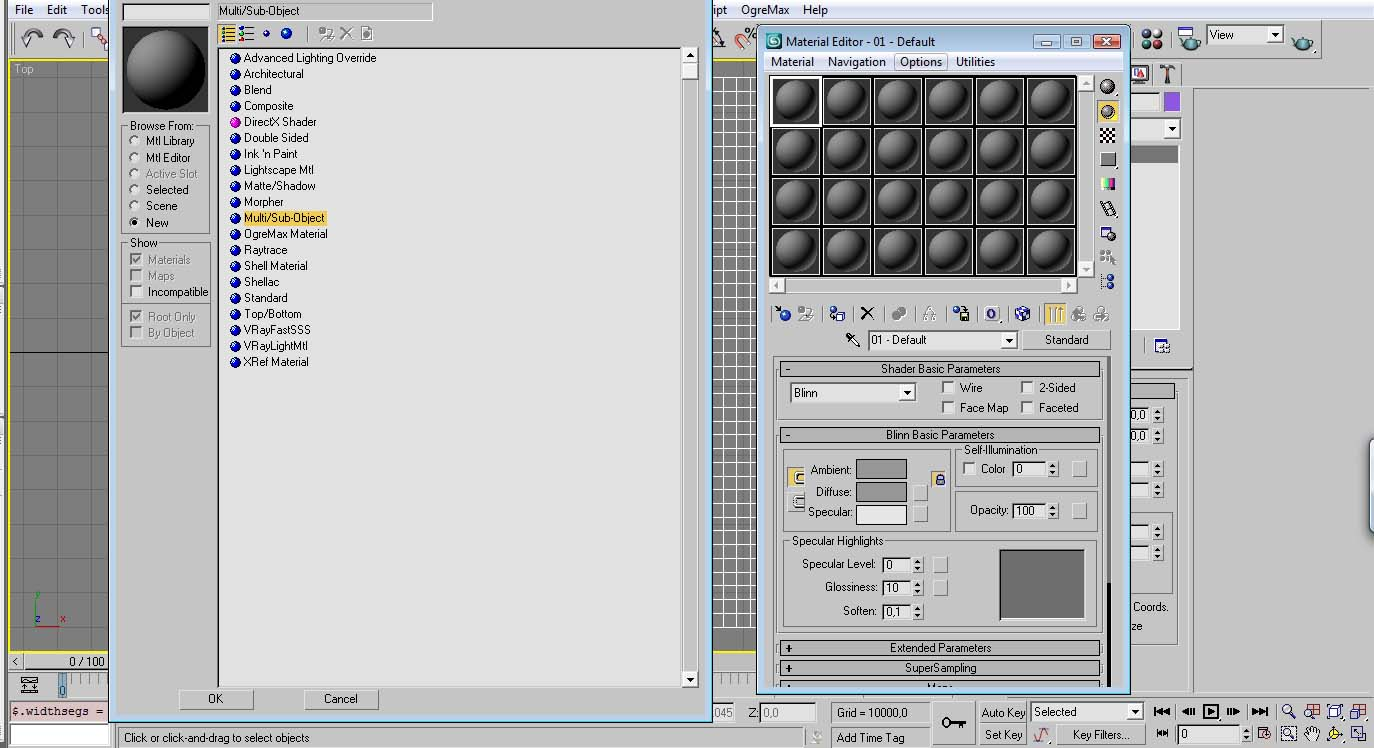In order to better organize the materials it is recommended to be compiled in a single “Multi/sub-Object”
Press the “Standard” button and then chose “Multi/sub-Object”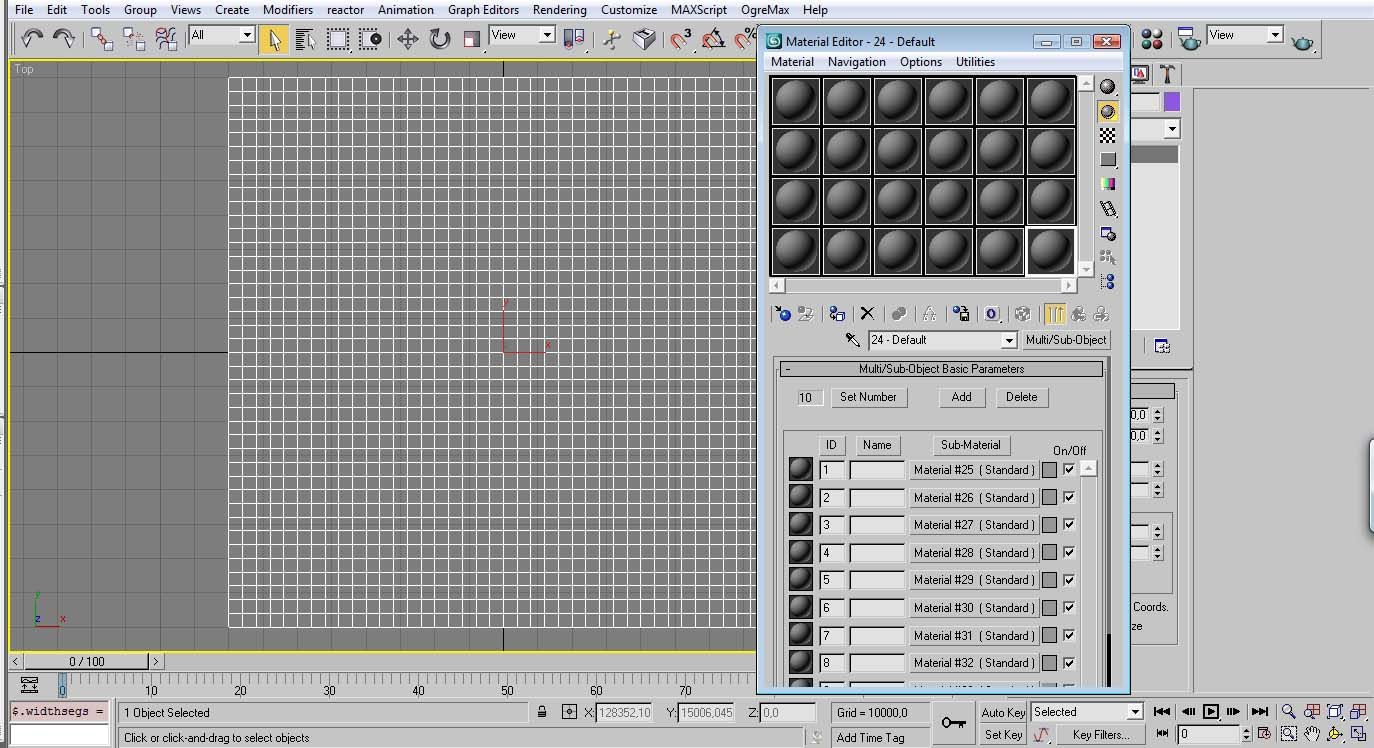Then chose the number of textures you may require by choosing in the “Set Number” button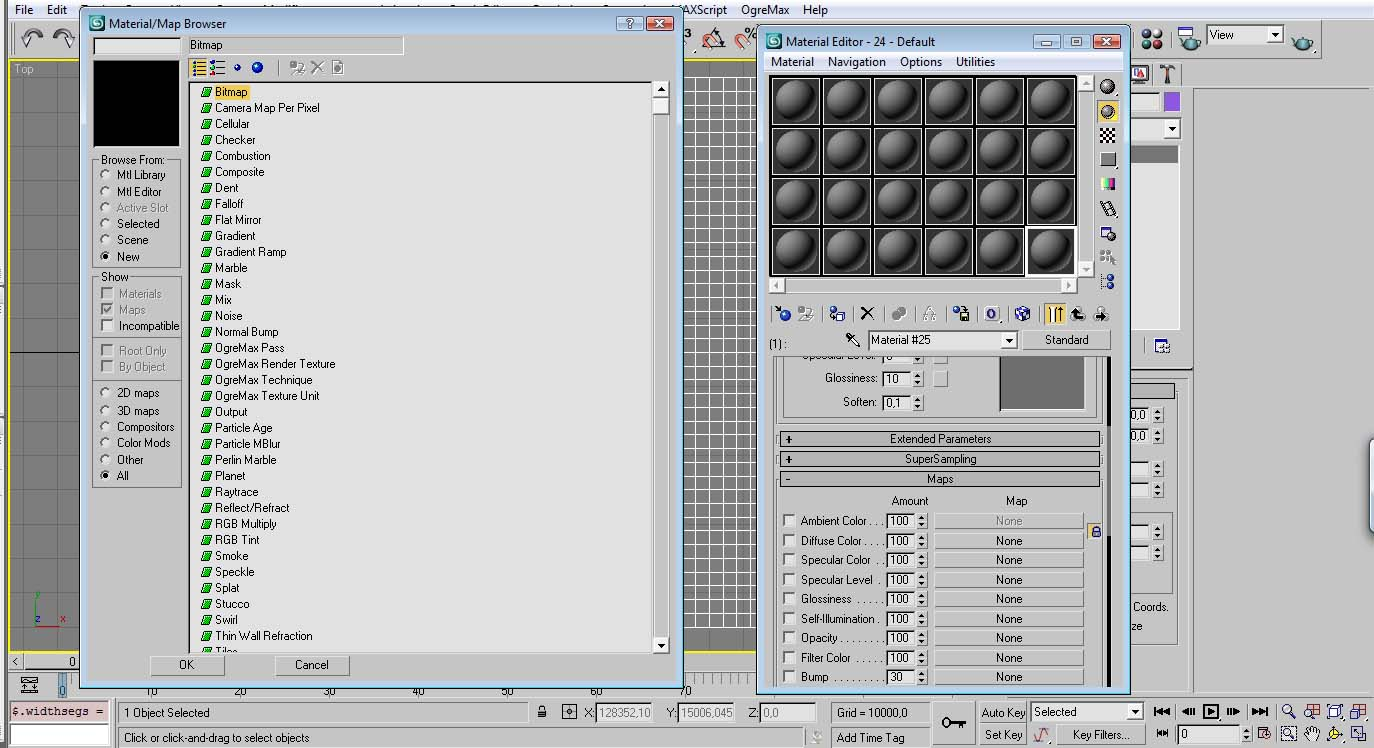After that click in the first material file and apply a bitmap file for the diffuse map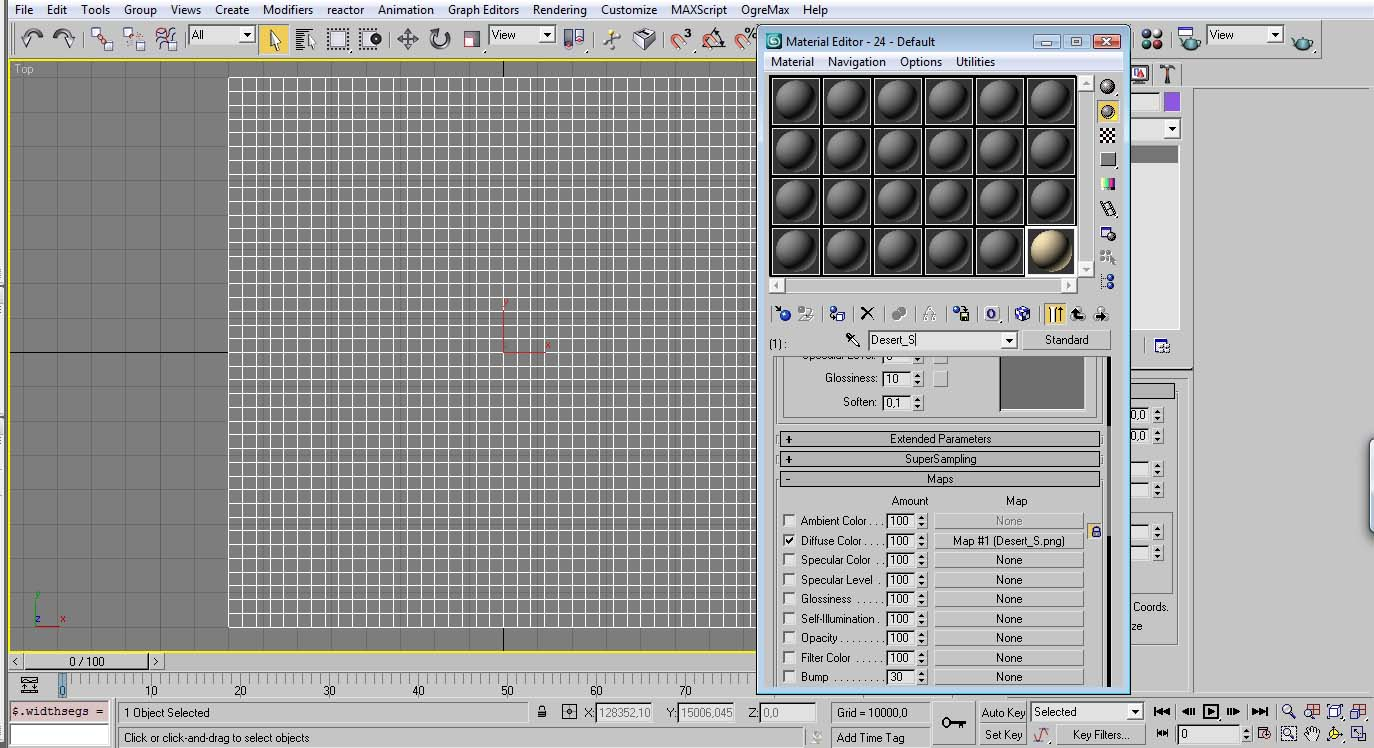You can find these textures in the game folder ..\media\Tilesets\
And preferably set the material name the same as the texture.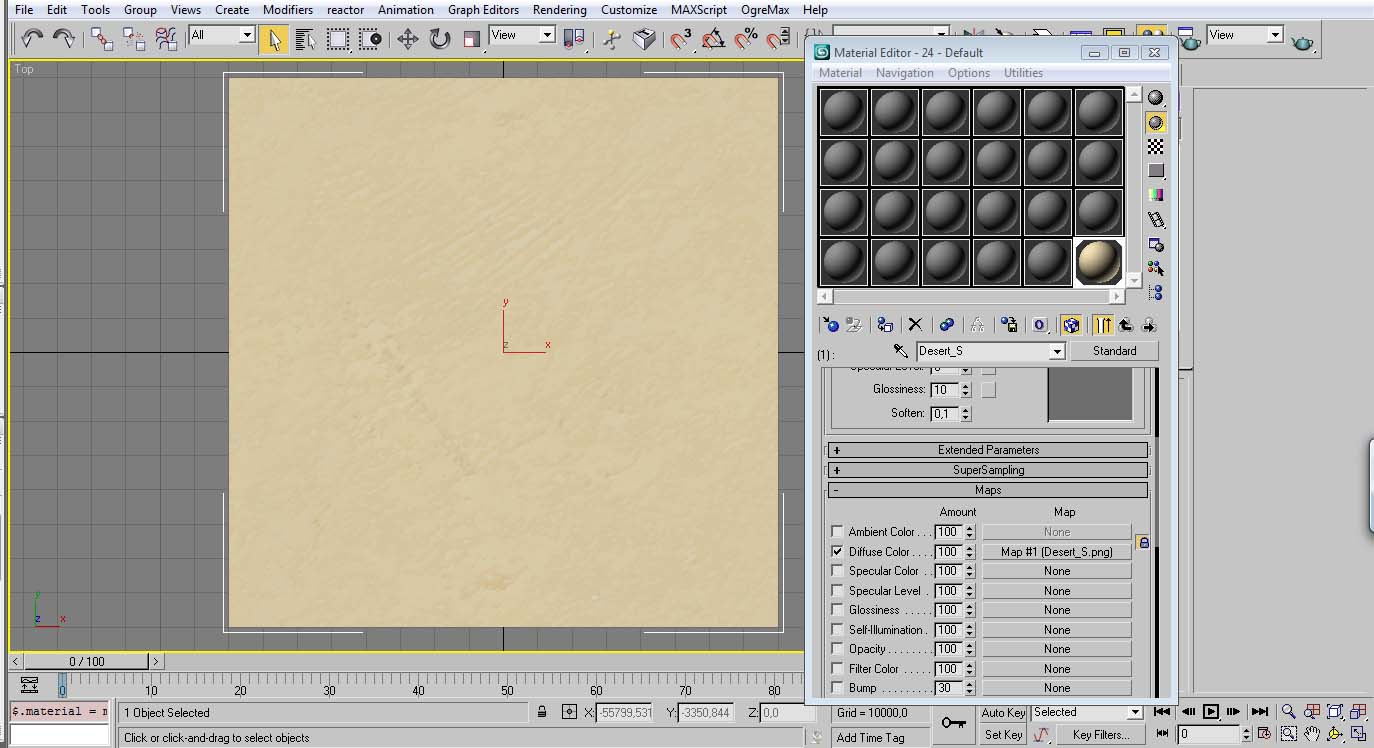Apply the material to the mesh.
(It is not visible in this image, but it is recommended to duplicate the new material as an instance to other sphere in the material editor and apply it to the model from there)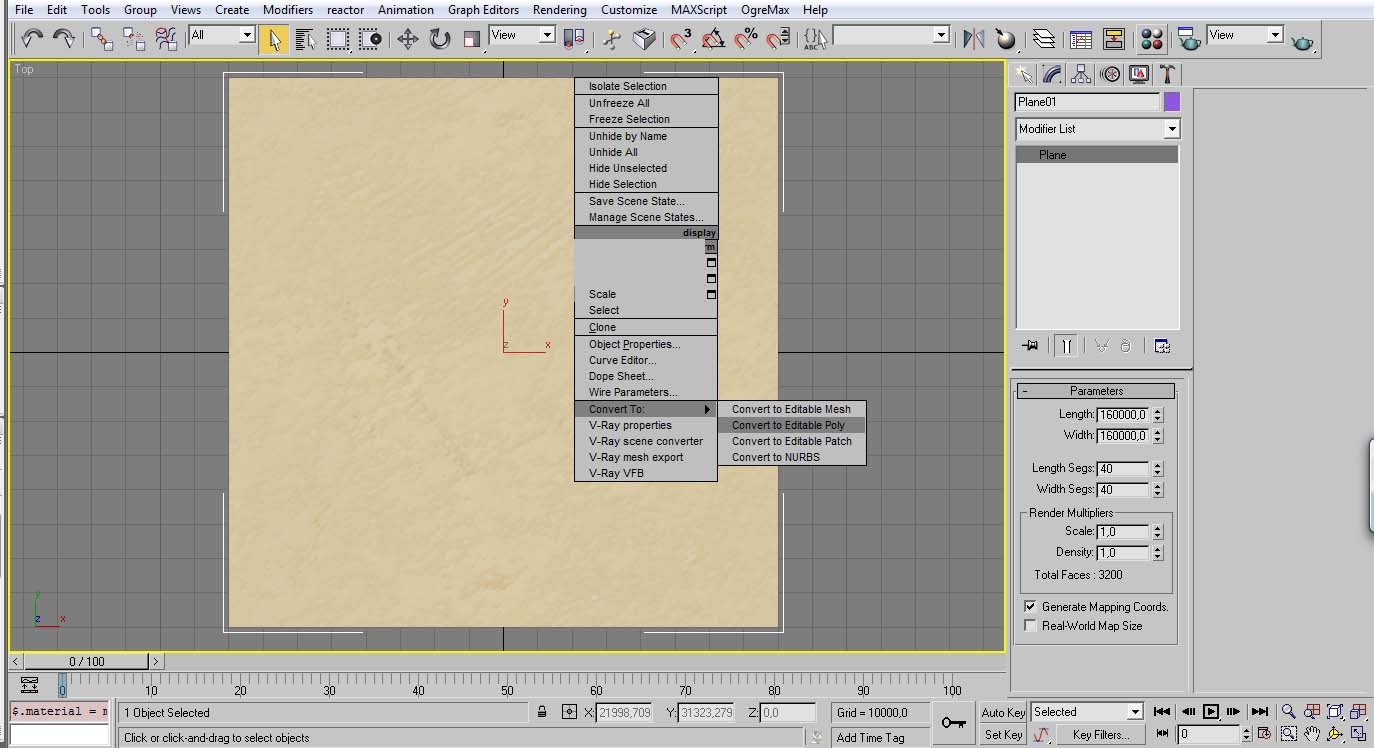Now convert the plane to an Editable Poly by clicking the right mouse button onto the mesh.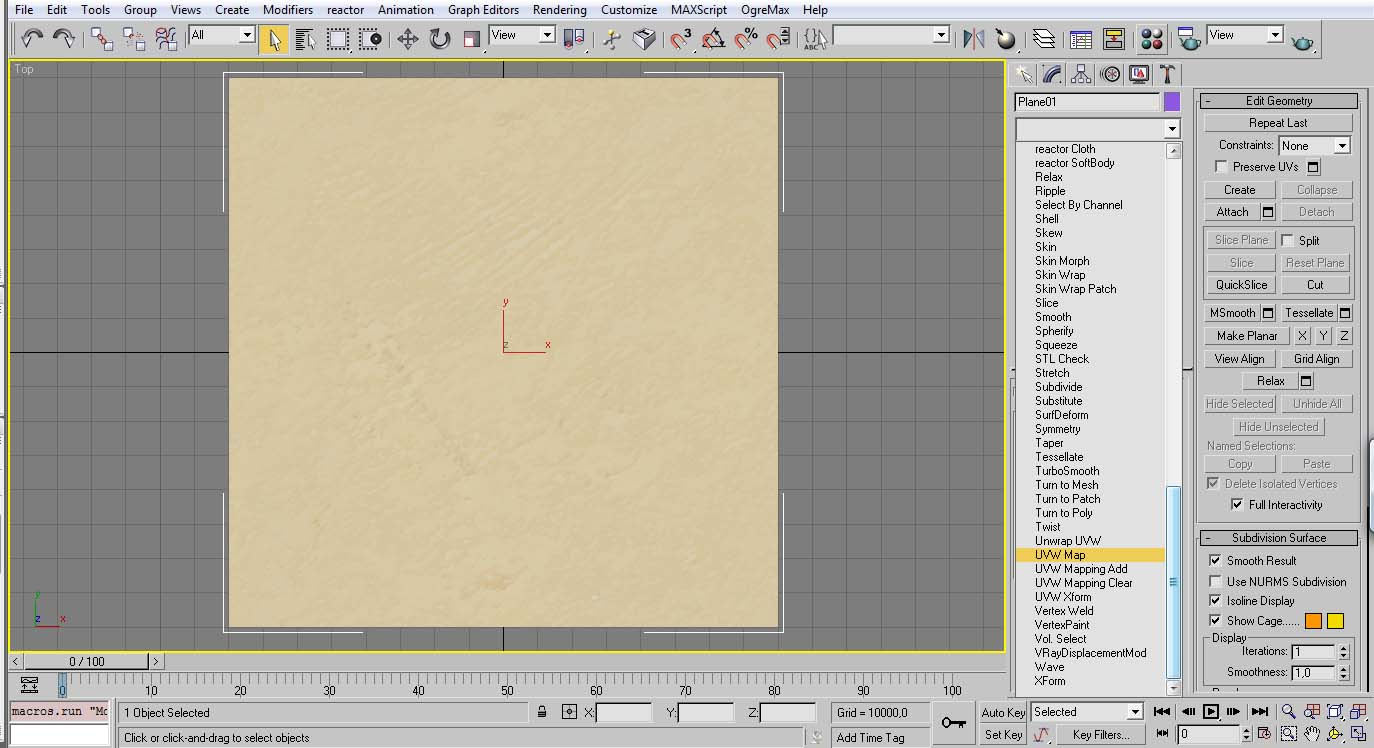Choose “UVW Map” in the modifier tab.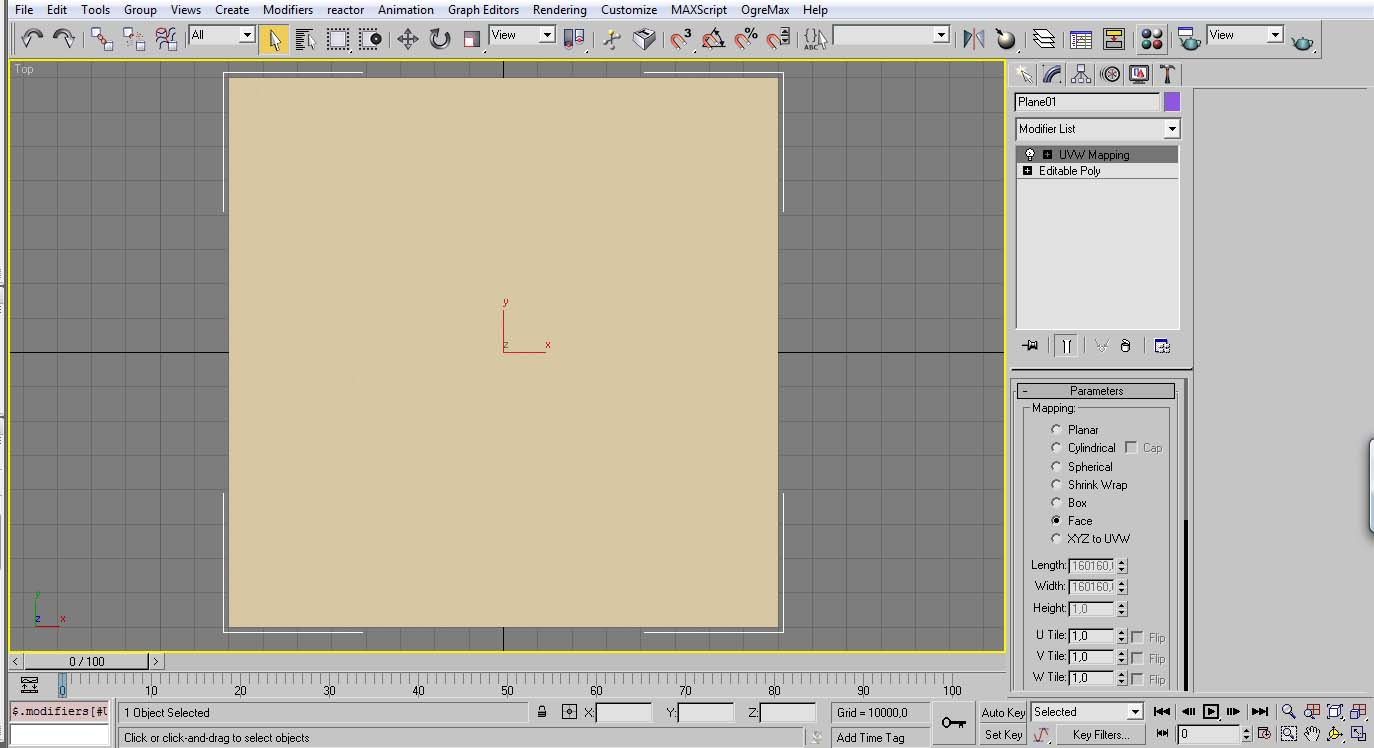Set the “Face” maping option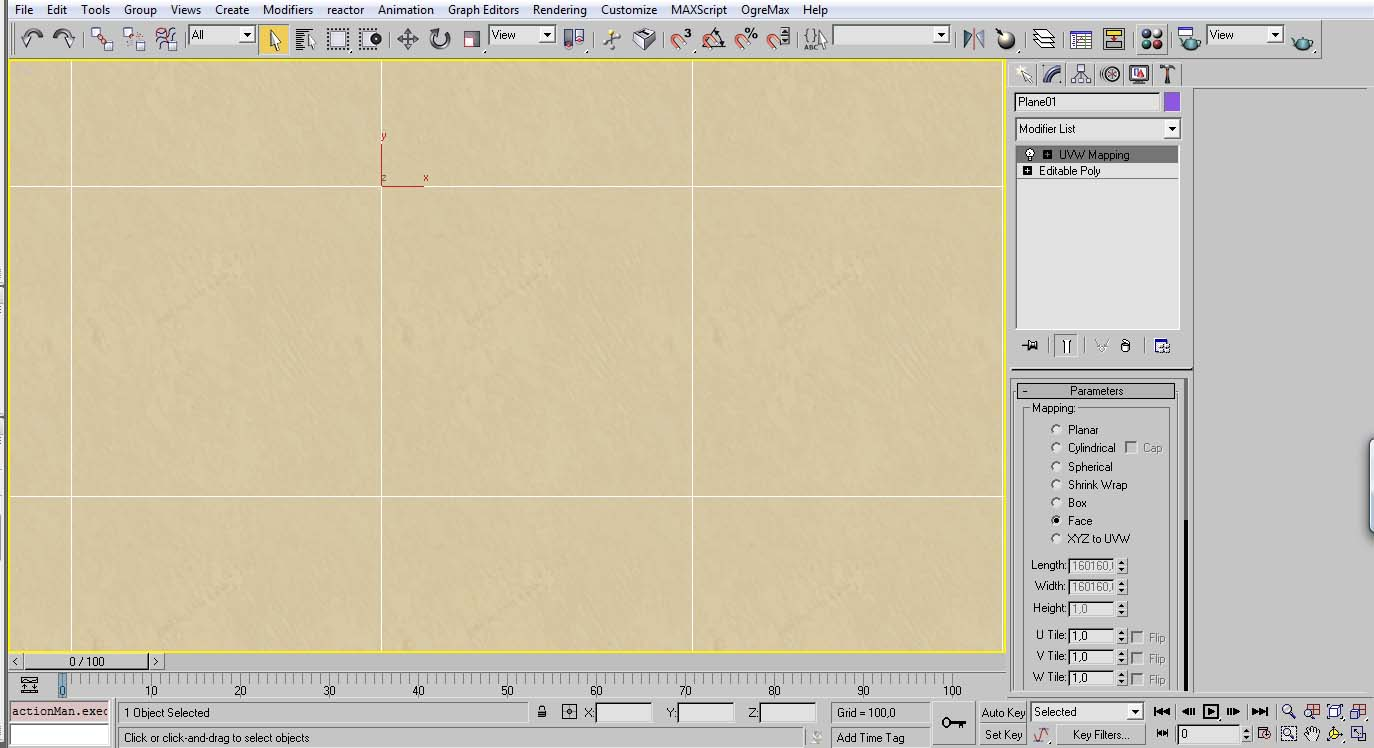As you can see the texture is proper applied to each square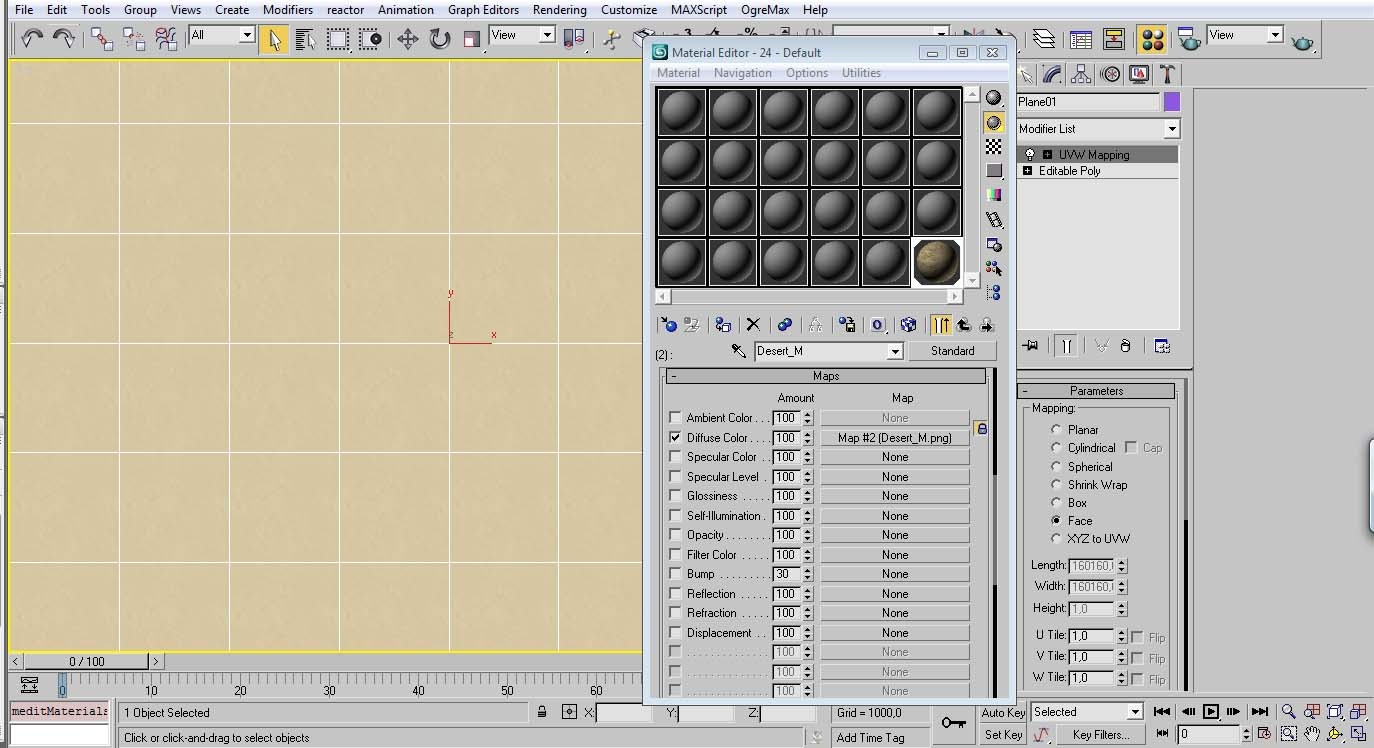Now and aditional texture was loaded, in this case was the mountain texture.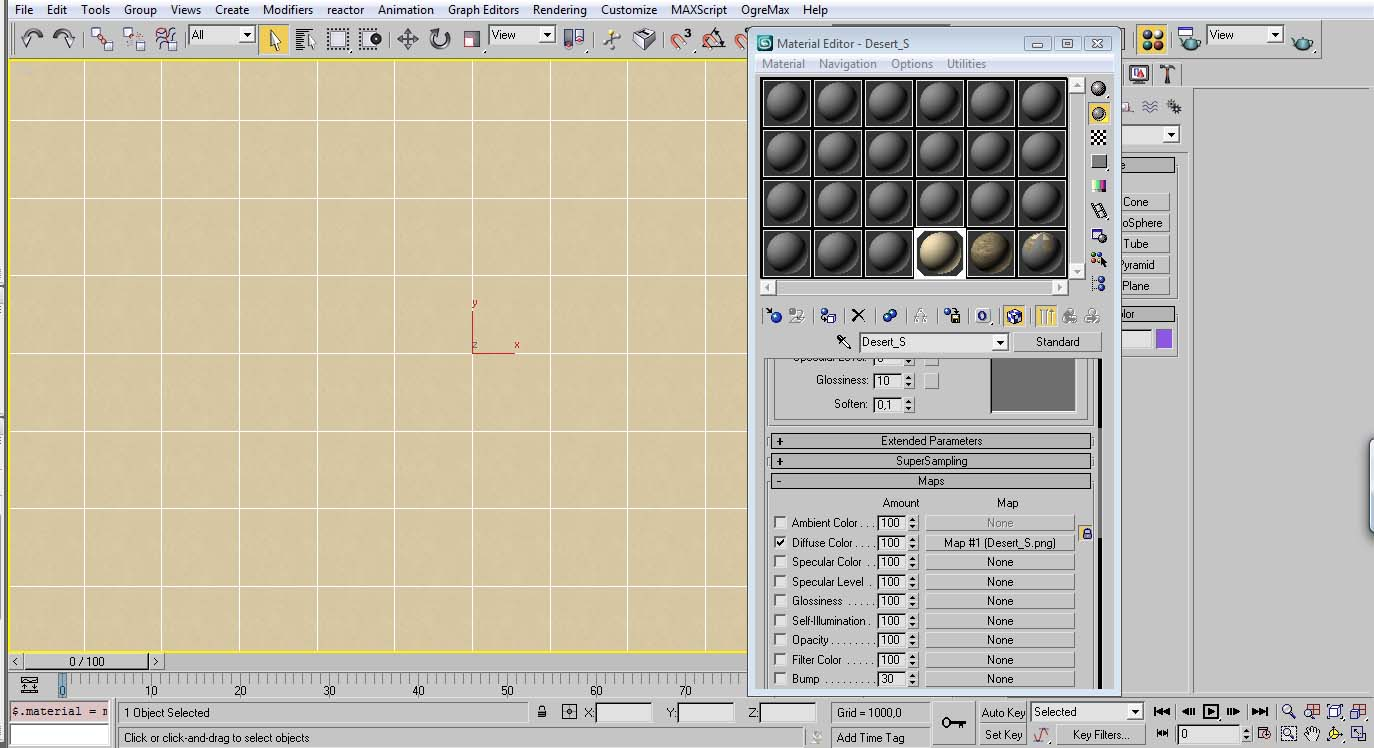Created instances of those materials like was mentioned above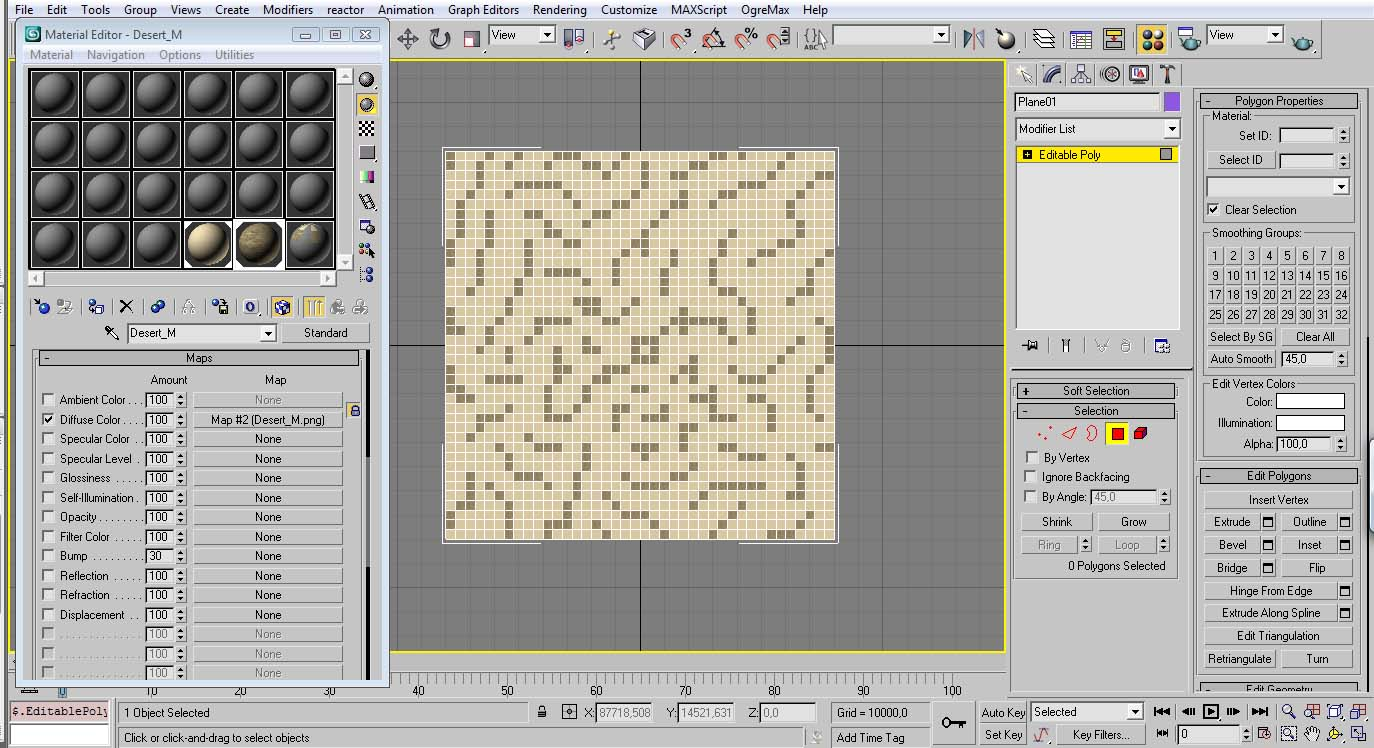The applied the texture where we wanted to make mountains. In this particular case the objective was to make a map with a high density in mountains.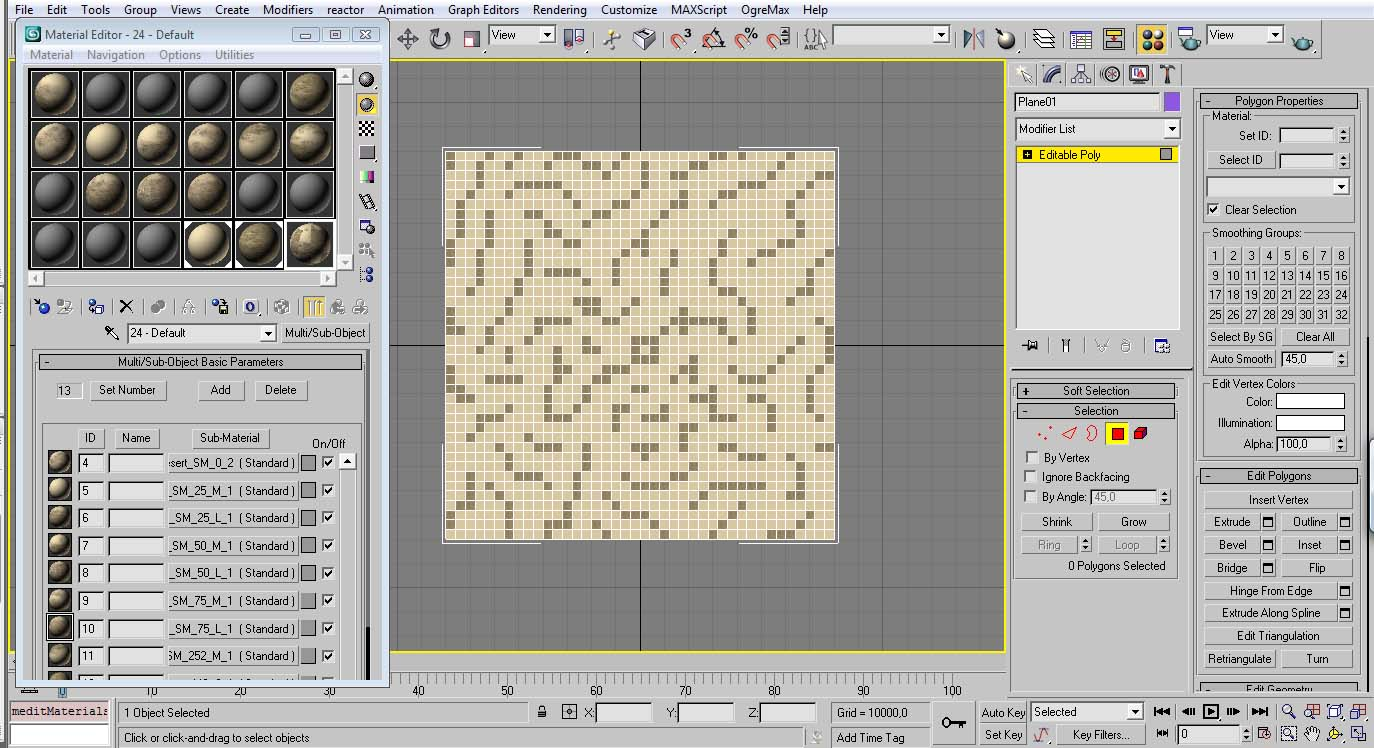After that, it was loaded all the transitions between the base material and the mountain texture and spread instances in the material editor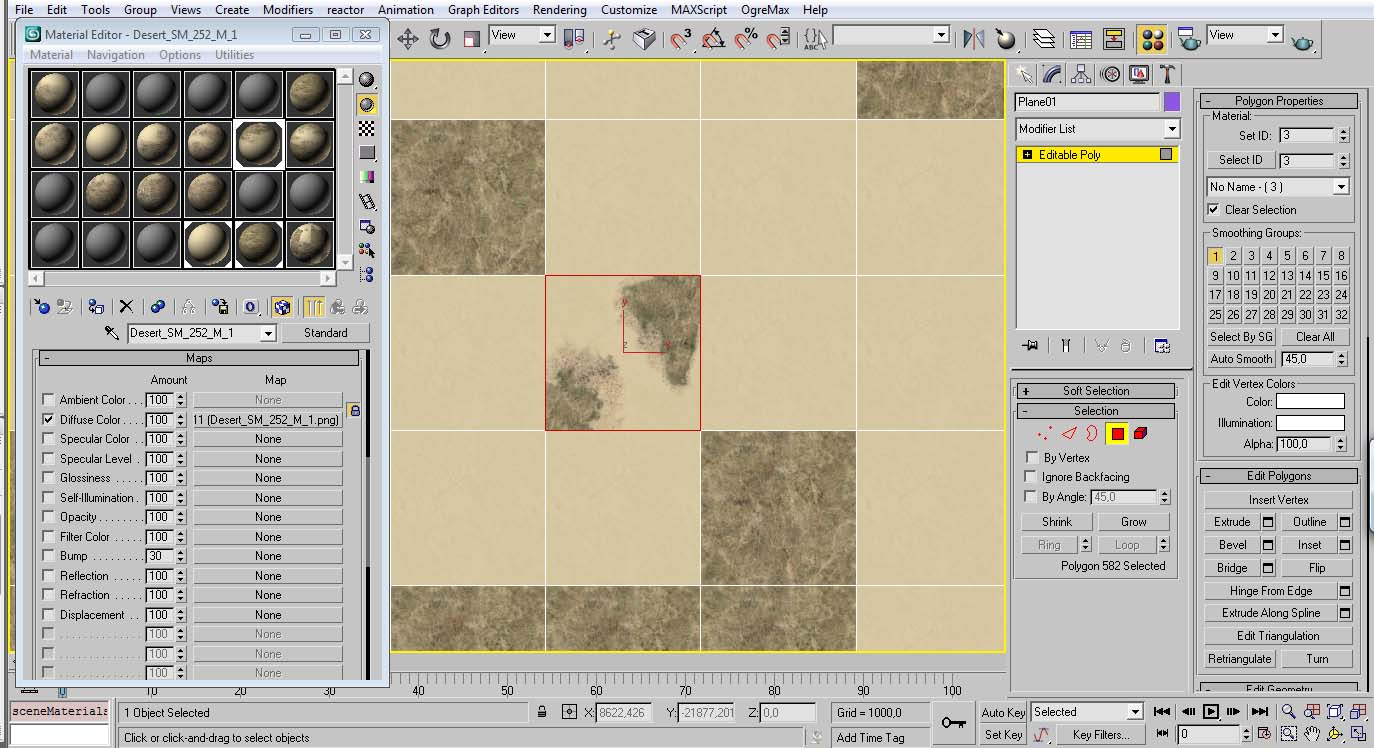Set a transition texture where you want.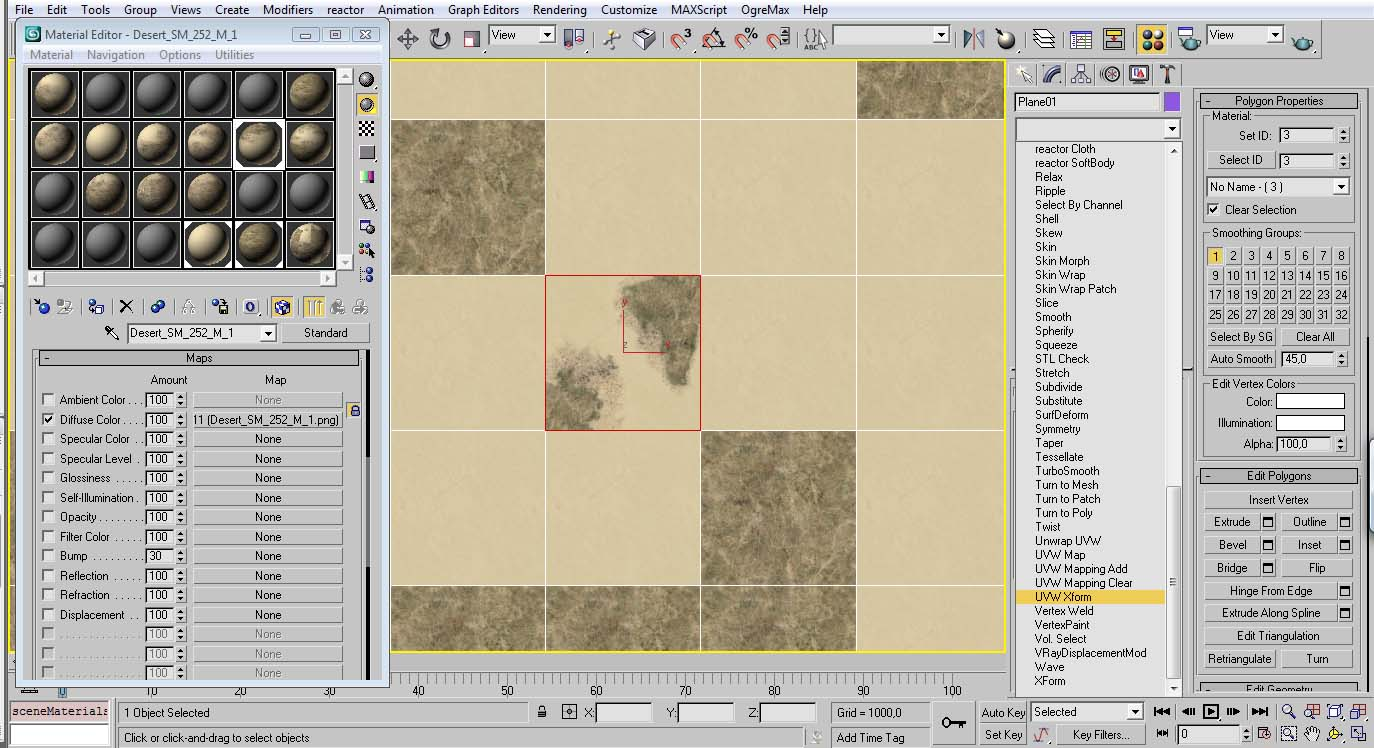Choose UVW Xform with that face selected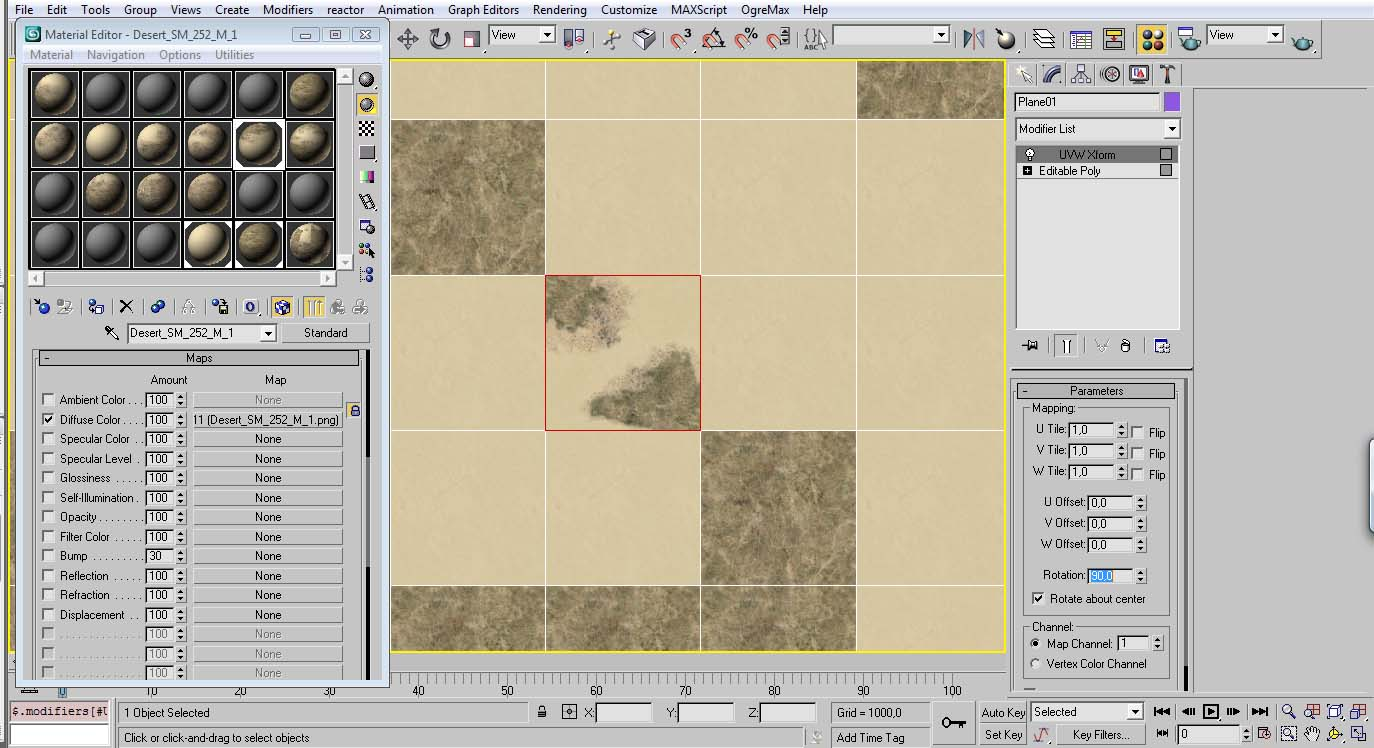And make the adjustments so the texture fit as you like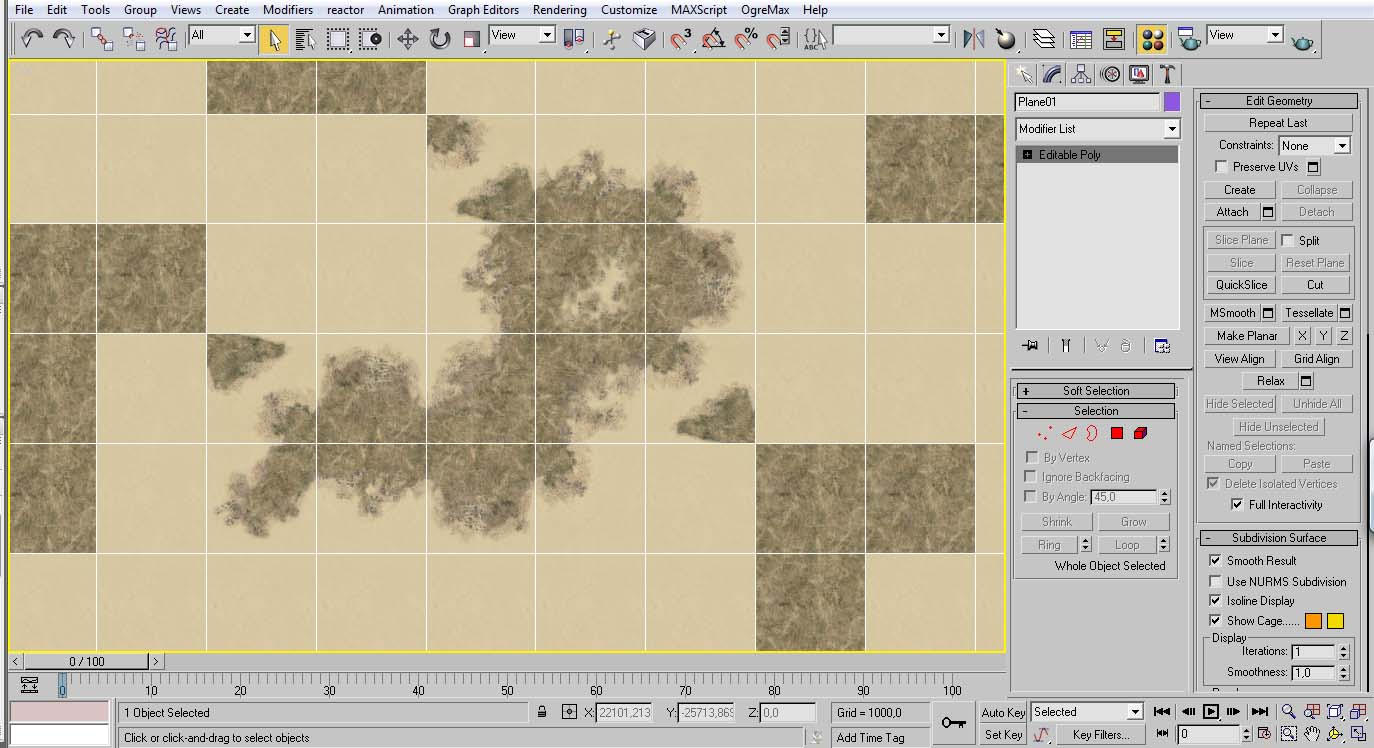Do the same to all other textures.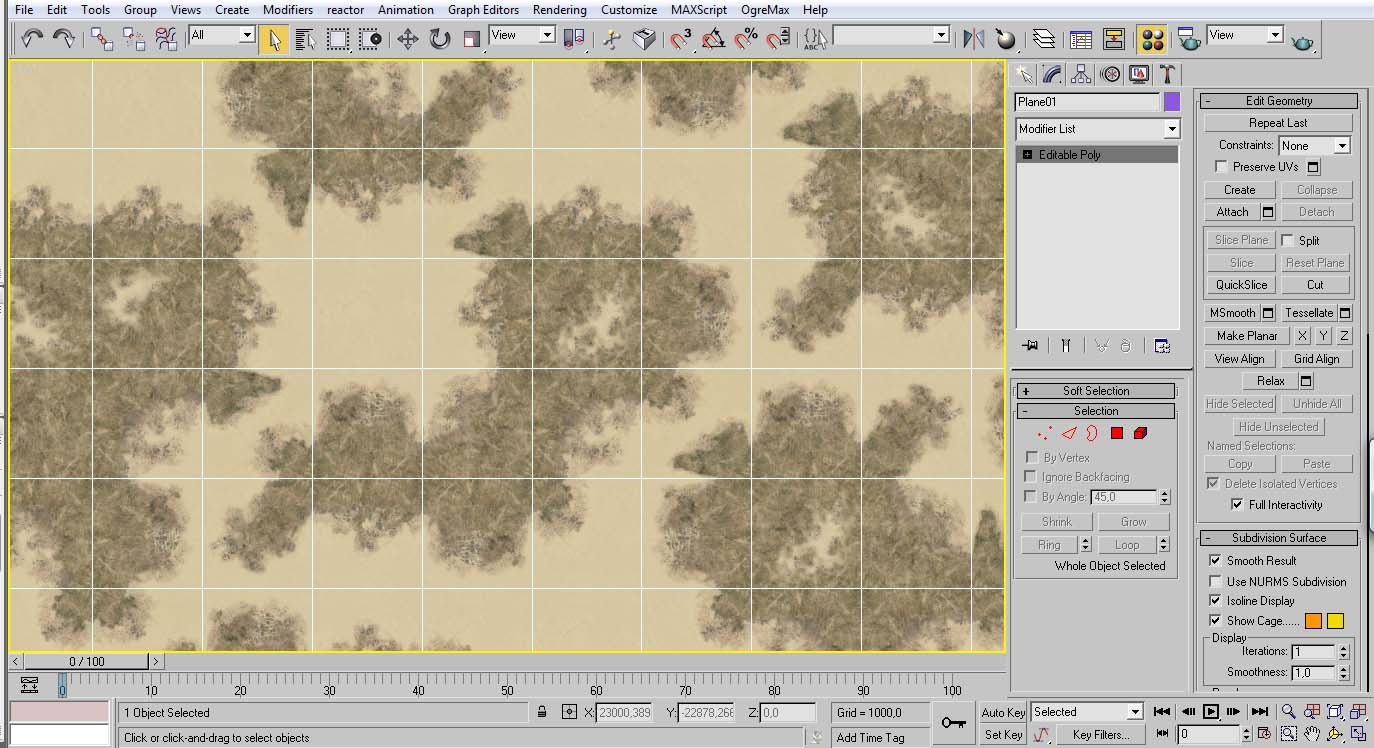A section of the map with all transitions done.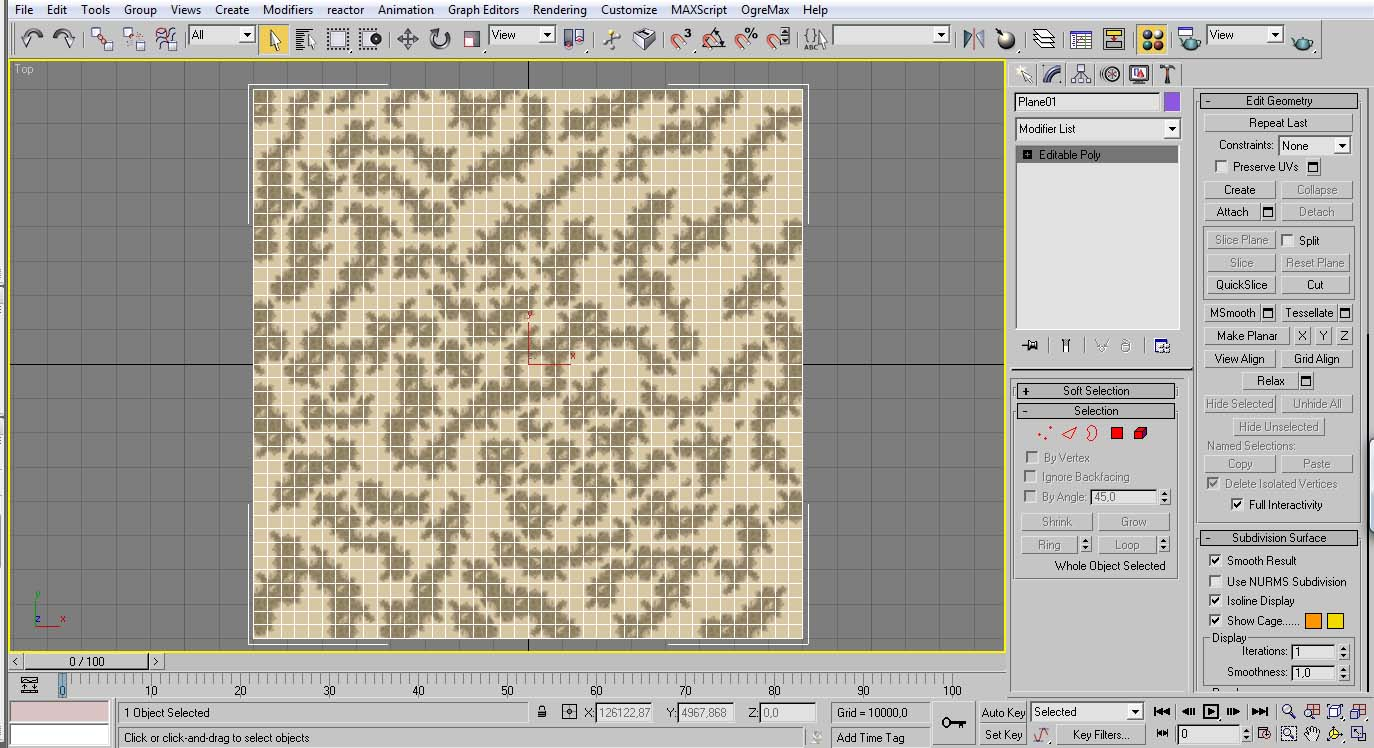And the entire map with the transitions compleated. (in your fist map it is recommended to have the less transitions as possible, depending how fast you are, applying these transitions can take some time)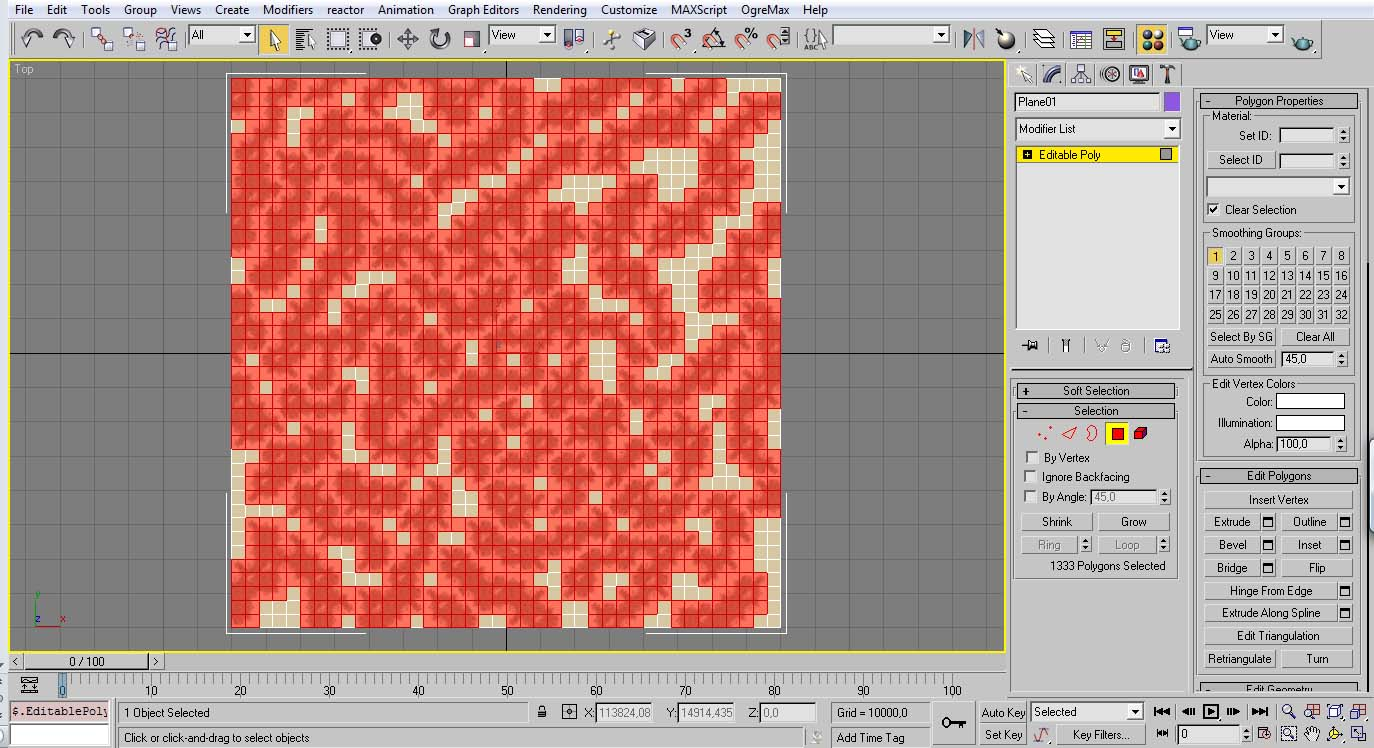Now select all tha faces with a mountain texture.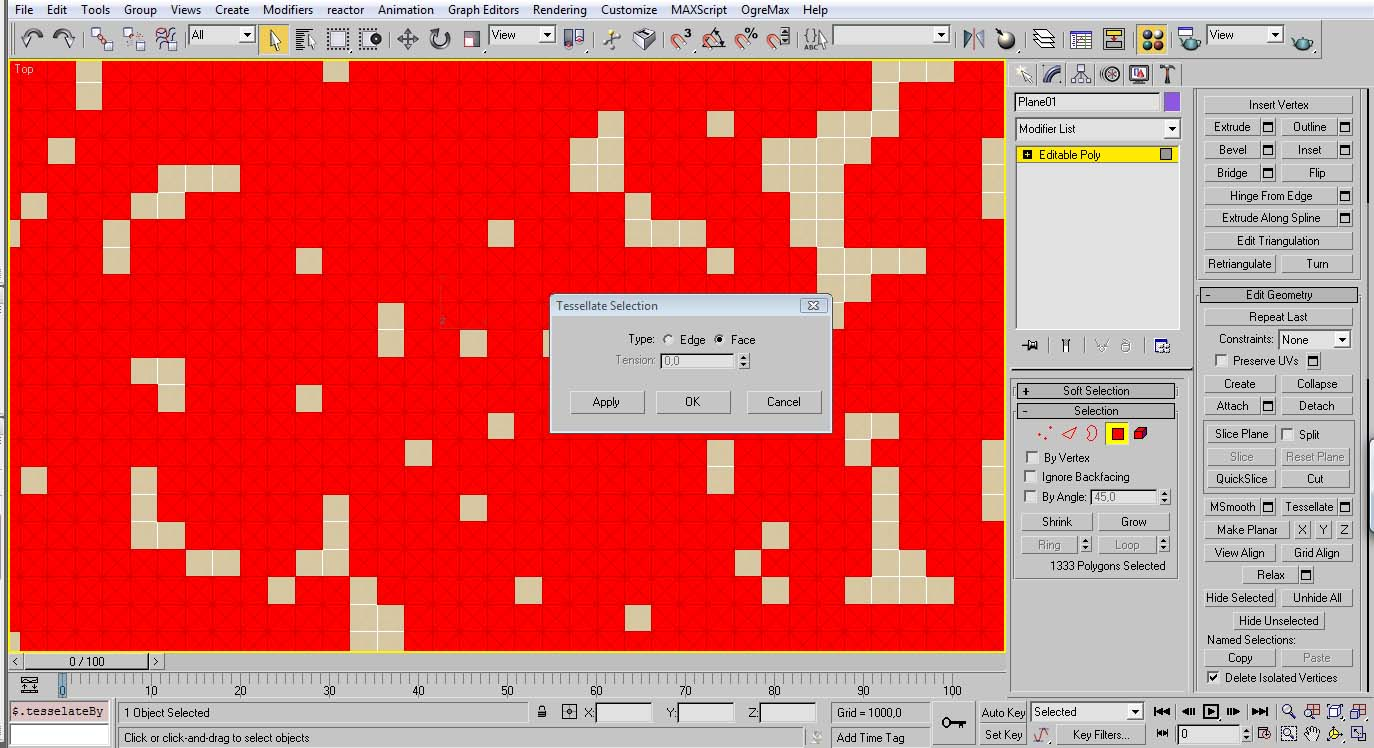Click in the button next to the tessellate button.
Choose face and apply.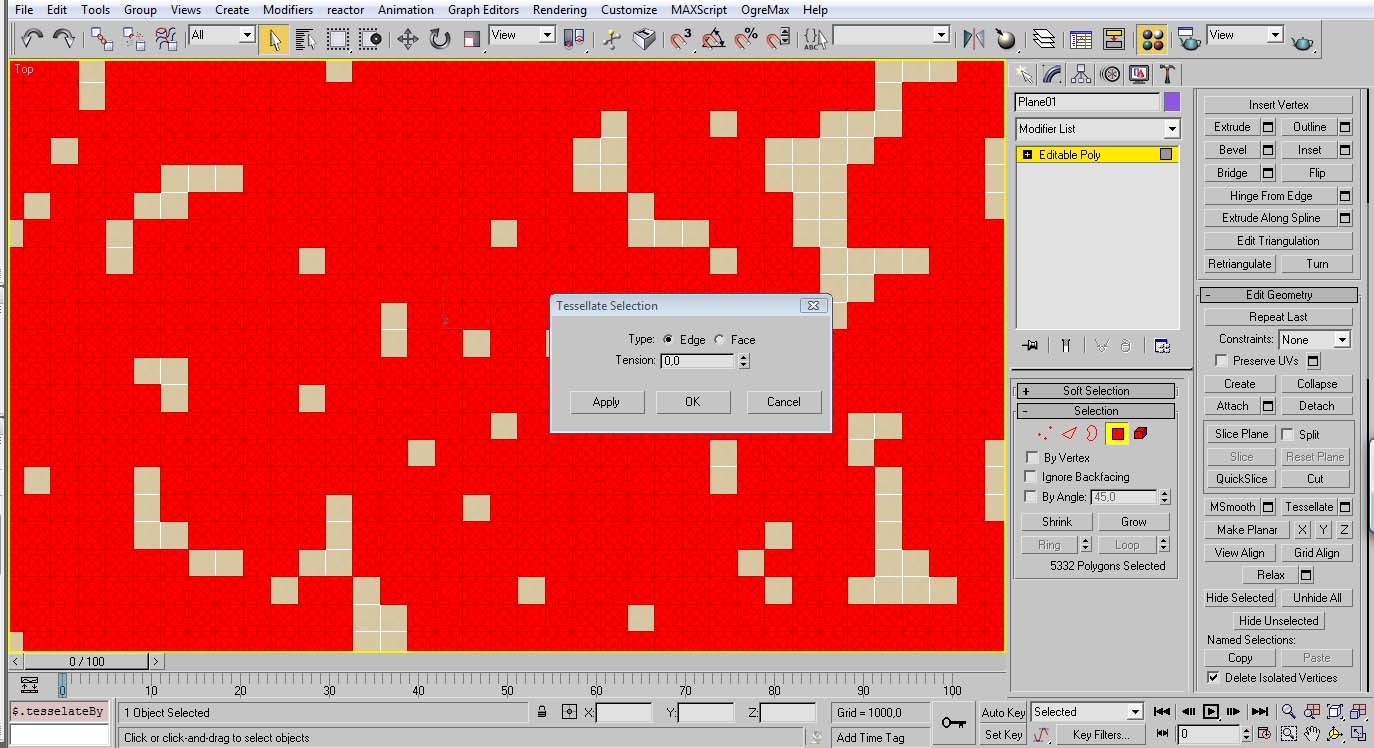Then do it again, but now choose edge.
This is to create enough sub divisions for the height displacement. These procedure is recommended because is quick and simple, and create subdivisions that fit nicely to the mountain shape. But you can use any other method that you like.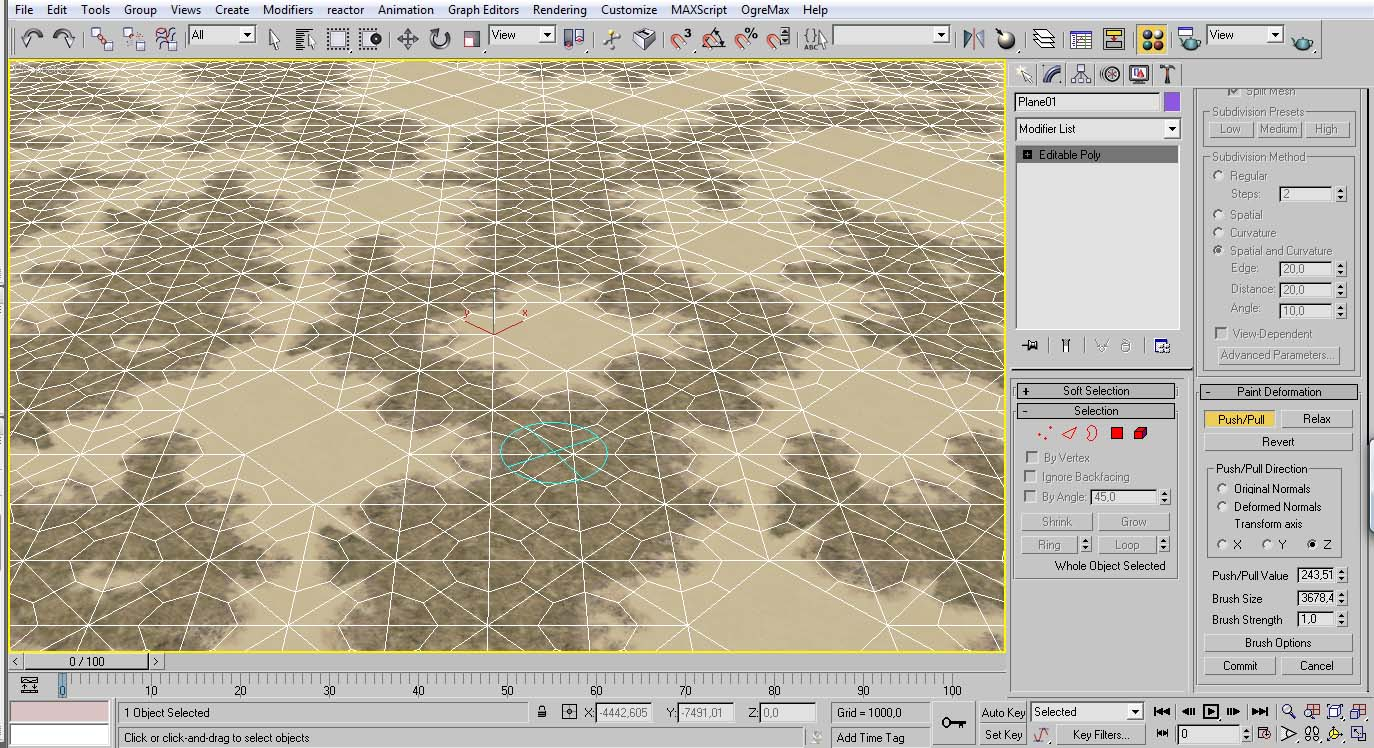Now, without having any face selected choose Push/Pull button and Z option.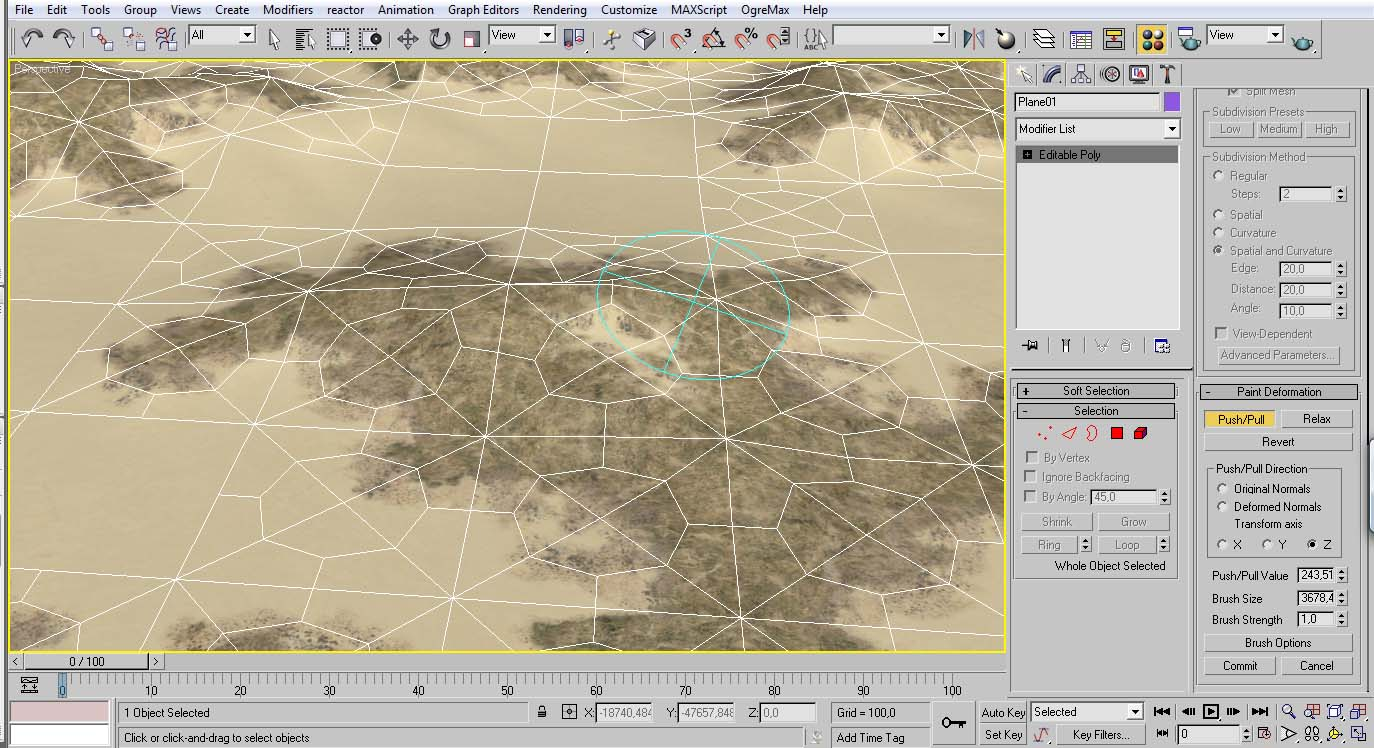Start shaping up your mountains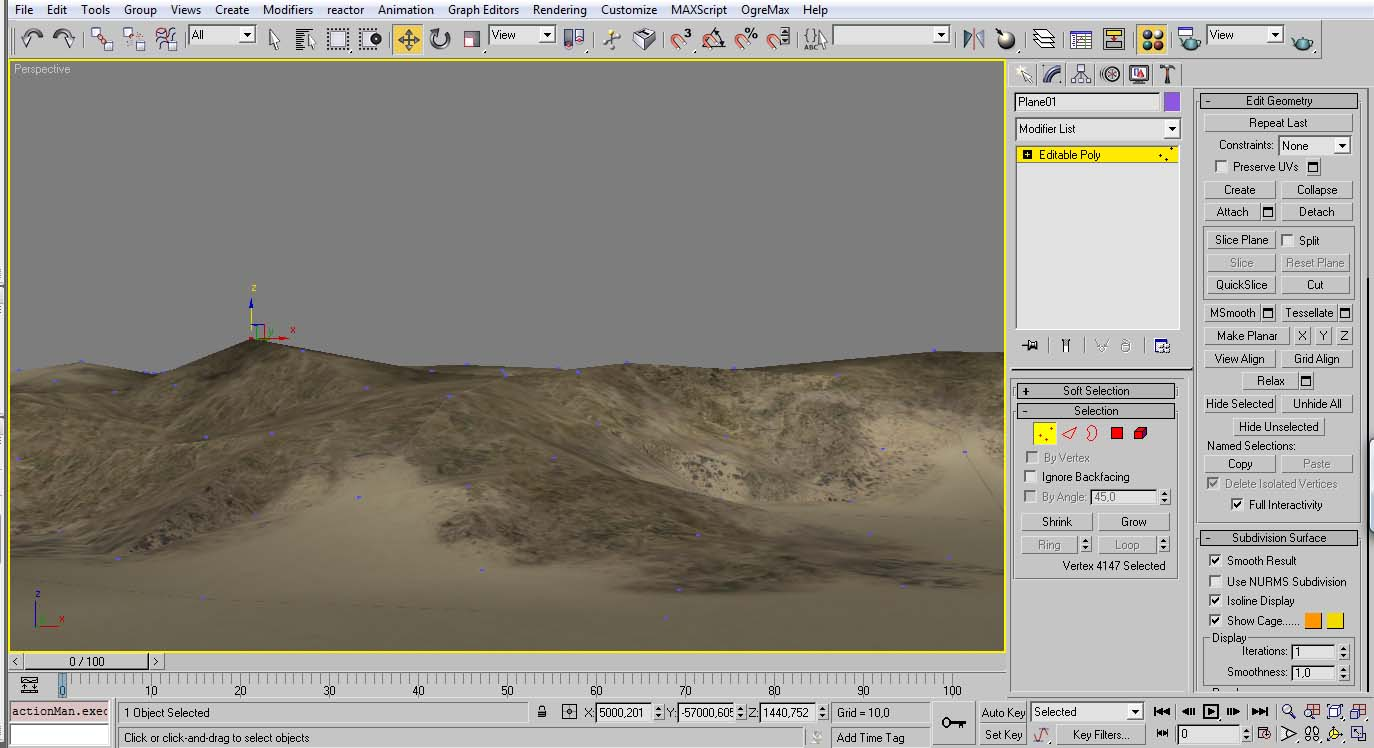It is still recommend to fine tune some points.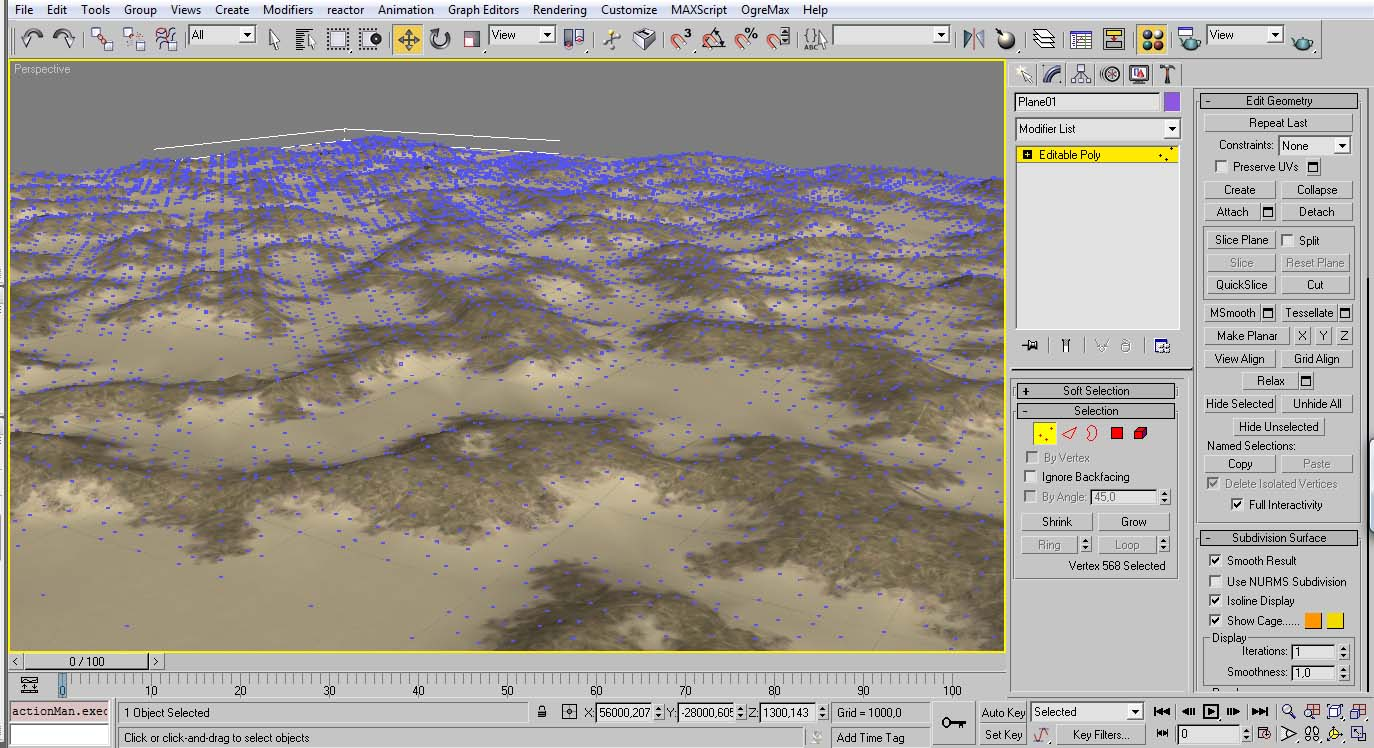Now with the volume the map is completed and we can now export it to the game.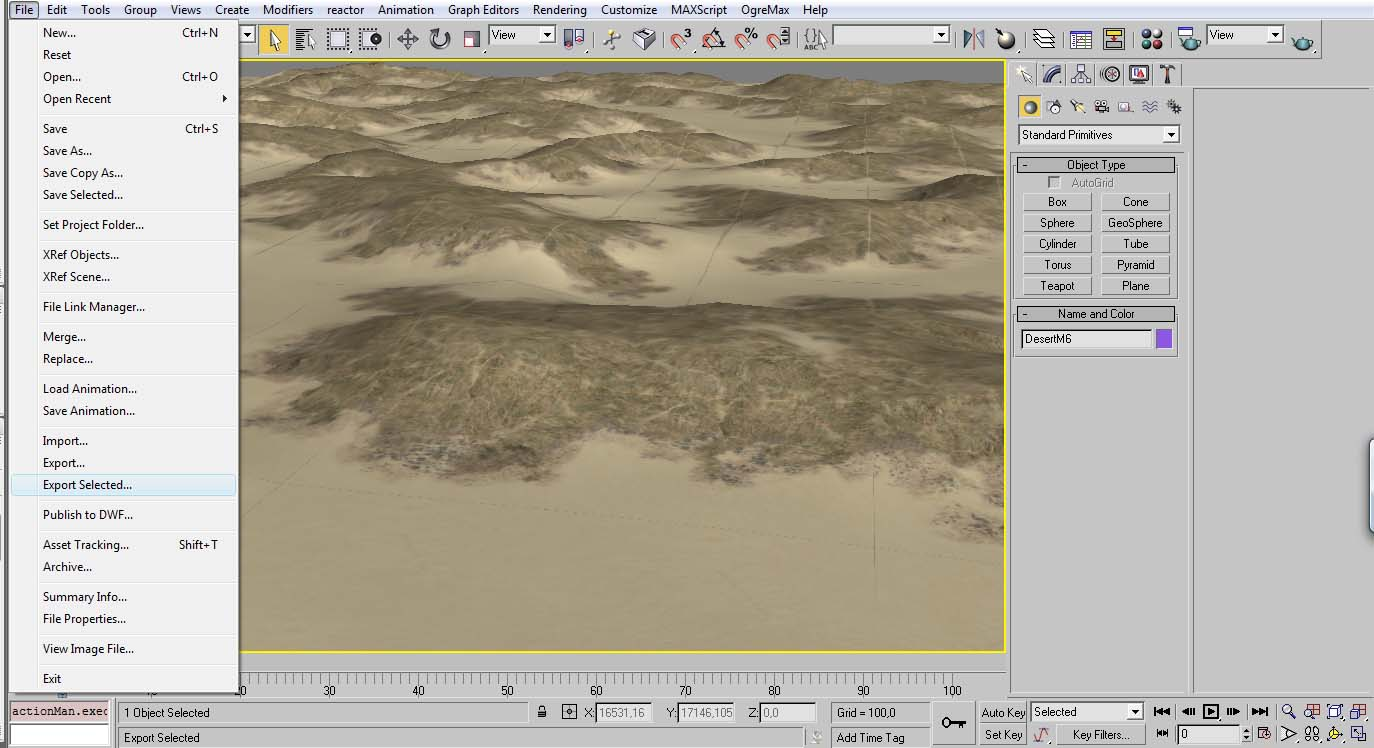Choose Export Selected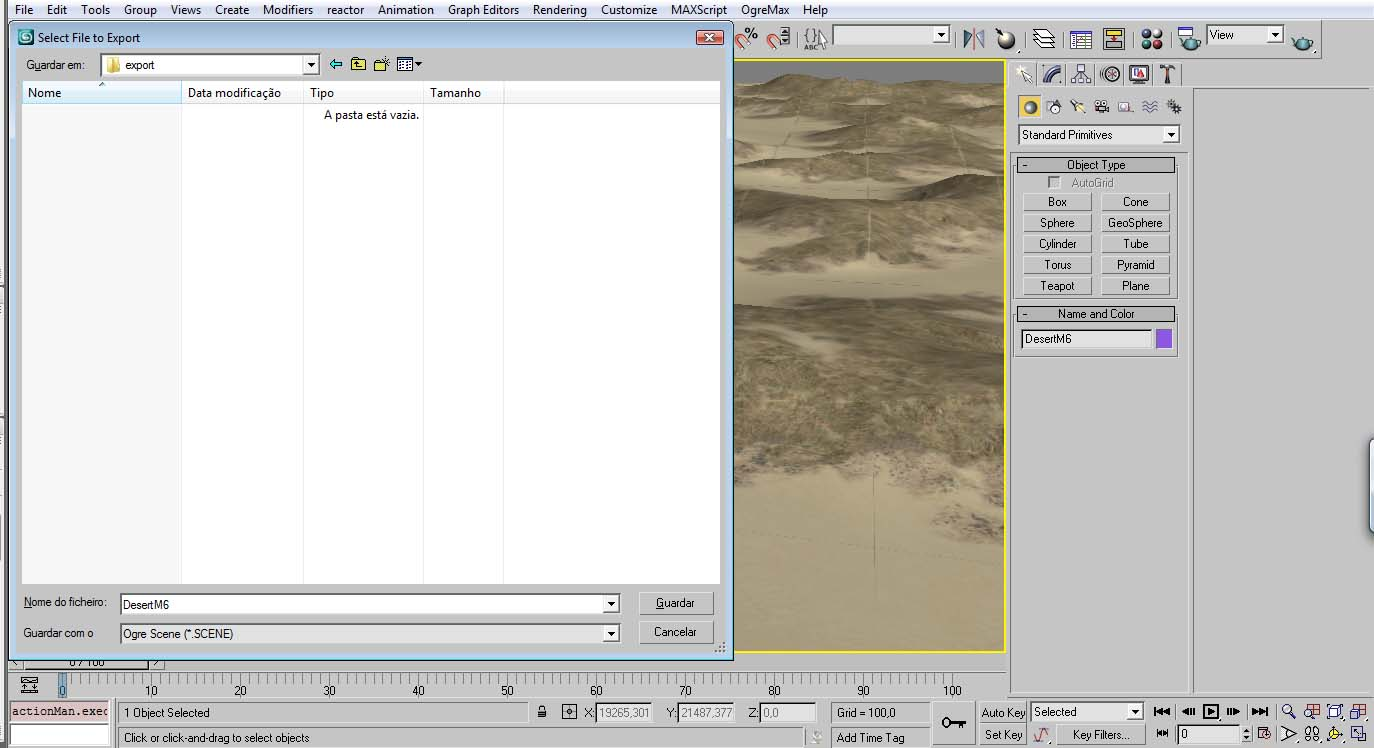Pick a folder (not a folder in the game)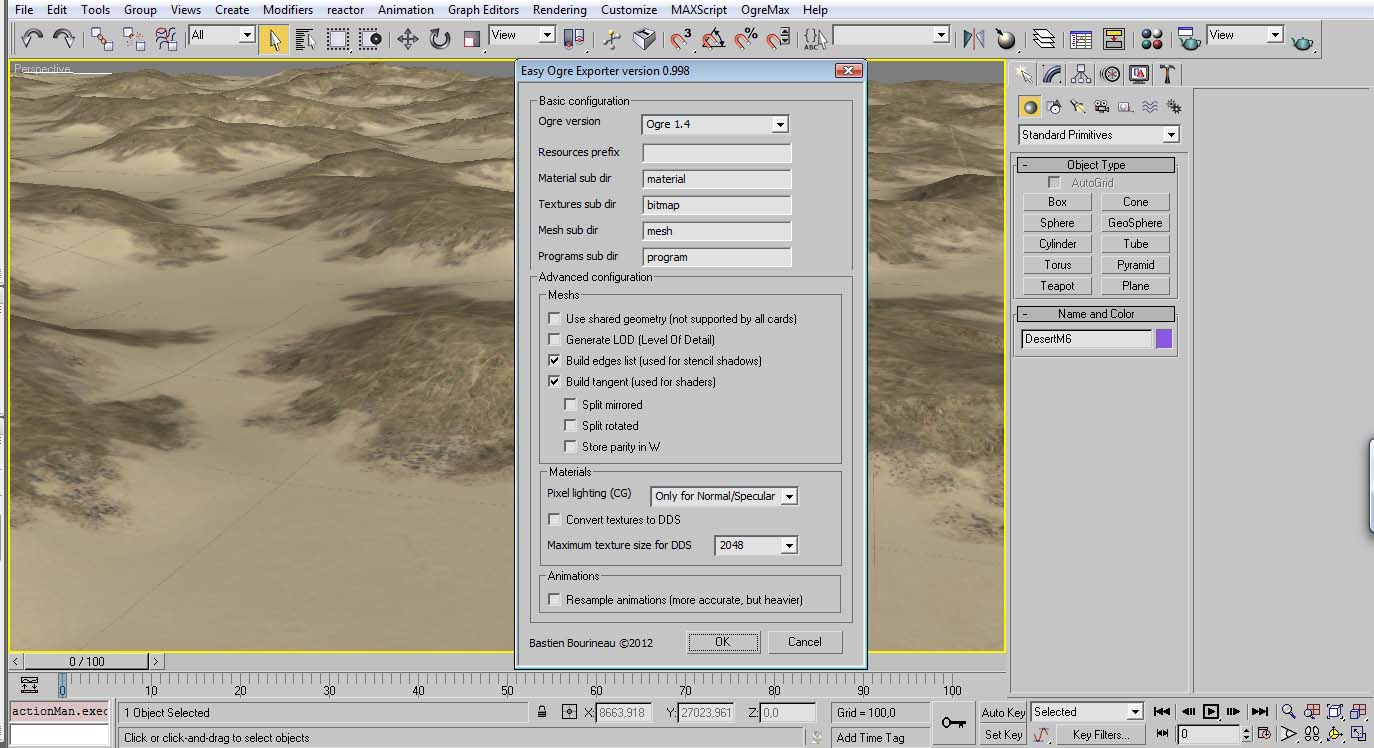Set the ogre version for 1.4 and export.
Then create a folder in the map folder of the game and place it there the exported mesh.

Create a Map.ini file and set the following parameters:

map1 code:
[scene]
...
nSNodes = "1"
nCollMesh= "1"

[Colisions]
Col_1_Mesh= "DesertM6.mesh"
Col_1_Pos=0
Col_1_TreeL_1_Prob=1.0

[sn_1]
sn_name="map1"
sn_IsSubNode = "false"
sn_ParentSn_name = "Root"
usa_eixos_3dMax="false"
sn_Pos="00 0 0"
sn_Yaw="0"
sn_Pitch="00"
sn_Roll="00"
sn_nMeshes= "1"
snMesh_Me_1 = "DesertM6.mesh
snMesh_Vis_1 = true
snMesh_UseCustomMat_1 = "false"
snMesh_CustomMatName_1 =

These are the most important for the game to load the mesh. For the collision it is using the same mesh as the original because the mesh is simple and doesn’t have any relevant difference in terrain, like a river or a forest.
However for high poly terrains is recommend to break in in pieces and place them individually.

And the completed map.ini file was set like this:

map1 code:
MapFile = ""

[scene]
AmbientLight = "0.3 0.3 0.3"
ShadowColour = "0.3 0.3 0.3"
// 1 ->skyX
// 1 ->skybox
// 2 ->skydome
TypeBG = "0"
BGmaterial = "Examples/CloudyNoonSkyBox"

NoFog = "false"
FogFadeColour = "0.78 0.698 0.60"
FogFadeColourAltitude = "0.76 0.76 0.80"
FogFadeKeyPoints = "500 7000"
FogStart = "10000"

NoSun = "false"
hasSun = "true"
Sun_AutoConfig = true
Sun_AutoConfig_Hour = true
Sun_position = "1500 1750 1300"
//Sun_position = "0 300 0"
Sun_DiffuseColour = "0.9 0.9 0.9"
Sun_SpecularColour= "0.9  0.9 1"
Sun_LensFlare = ""

NoGroundLightScater  = "false"
hasGroundLightScater = "true"
dScaterLight_DiffuseColour = "0.06 0.06 0.08"
ScaterLight_DiffuseColour = "0.2 0.15 0.05"
ScaterLight_SpecularColour= "0.16  0.1 0.06"

nDirectLights = "0"
nSNodes = "1"
nCollMesh= "1"
StaticGeom="0"

[Clouds]
numLayers=9

Clayer_1_type=1
Clayer_1_Ypos=6000
Clayer_1_Texture= "al_pClfog.png""
Clayer_1_ModelType=0

Clayer_2_type=4
Clayer_2_Ypos=4000
Clayer_2_YvarRange=6000
Clayer_2_Texture="al_bCloud4.png
Clayer_2_BillType = 2
Clayer_2_NumClouds=150
Clayer_2_BillDefDimen = "3000 3000"

Clayer_3_type=2
Clayer_3_Ypos=1000
Clayer_3_Texture= "al_cCloud.png"
Clayer_3_ModelType=0

Clayer_4_type=3
Clayer_4_Ypos=6000
Clayer_4_Texture= "al_dCloud.png"
Clayer_4_ModelType=0

Clayer_5_type=4
Clayer_5_Ypos=3000
Clayer_5_YvarRange=4000
Clayer_5_Texture="DistBCloud1.png"
Clayer_5_BillType = 1
Clayer_5_NumClouds=200
Clayer_5_BillDefDimen = "4000 1000"

Clayer_6_type=4
Clayer_6_Ypos=4000
Clayer_6_YvarRange=4000
Clayer_6_Texture="al_bCloud1.png
Clayer_6_BillType = 1
Clayer_6_NumClouds=200
Clayer_6_BillDefDimen = "4000 2000"

Clayer_7_type=4
Clayer_7_Ypos=4000
Clayer_7_YvarRange=4000
Clayer_7_Texture="al_bCloud2.png
Clayer_7_BillType = 1
Clayer_7_NumClouds=150
Clayer_7_BillDefDimen = "2000 1000"

Clayer_8_type=4
Clayer_8_Ypos=6000
Clayer_8_YvarRange=8000
Clayer_8_Texture="al_bCloud3.png
Clayer_8_BillType = 2
Clayer_8_NumClouds=100
Clayer_8_BillDefDimen = "10000 5000"

Clayer_9_type=4
Clayer_9_Ypos=4000
Clayer_9_YvarRange=6000
Clayer_9_Texture="al_bCloud3.png
Clayer_9_BillType = 2
Clayer_9_NumClouds=200
Clayer_9_BillDefDimen = "4000 4000"

[Colisions]
Col_1_Mesh= "DesertM6.mesh"
Col_1_Pos=0
Col_1_TreeL_1_Prob=1.0

[sn_1]
sn_name="map1"
sn_IsSubNode = "false"
sn_ParentSn_name = "Root"
usa_eixos_3dMax="false"
sn_Pos="00 0 0"
sn_Yaw="0"
sn_Pitch="00"
sn_Roll="00"
sn_nMeshes= "1"
snMesh_Me_1 = "DesertM6.mesh
snMesh_Vis_1 = true
snMesh_UseCustomMat_1 = "false"
snMesh_CustomMatName_1 =

[TerrainMaterials]
NumMaterials = 30
DetailTexture = """
WaterTexture = ""
WaterToneOverlay = ""
TerrainToneOverlay= ""
OceanMatName= ""

Mat_1_MaterialName="Desert_M"
Mat_1_TextureName="Desert_M.png"
Mat_1_WasWater = false

Mat_2_MaterialName="Desert_MS_0_1"
Mat_2_TextureName="Desert_MS_0_1.png"
Mat_2_WasWater = false

Mat_3_MaterialName="Desert_MS_0_2"
Mat_3_TextureName="Desert_MS_0_2.png"
Mat_3_WasWater = false

Mat_4_MaterialName="Desert_S"
Mat_4_TextureName="Desert_S.png"
Mat_4_WasWater = false

Mat_5_MaterialName="Desert_SF_0_1"
Mat_5_TextureName="Desert_SF_0_1.png"
Mat_5_WasWater = false

Mat_6_MaterialName="Desert_SF_0_2"
Mat_6_TextureName="Desert_SF_0_2.png"
Mat_6_WasWater = false

Mat_7_MaterialName="Desert_SM_0_1"
Mat_7_TextureName="Desert_SM_0_1.png"
Mat_7_WasWater = false

Mat_8_MaterialName="Desert_SM_0_2"
Mat_8_TextureName="Desert_SM_0_2.png"
Mat_8_WasWater = false

Mat_9_MaterialName="Desert_SM_252_M_1"
Mat_9_TextureName="Desert_SM_252_M_1.png"
Mat_9_WasWater = false

Mat_10_MaterialName="Desert_SM_25_L_1"
Mat_10_TextureName="Desert_SM_25_L_1.png"
Mat_10_WasWater = false

Mat_11_MaterialName="Desert_SM_25_M_1"
Mat_11_TextureName="Desert_SM_25_M_1.png"
Mat_11_WasWater = false

Mat_12_MaterialName="Desert_SM_50_L_1"
Mat_12_TextureName="Desert_SM_50_L_1.png"
Mat_12_WasWater = false

Mat_13_MaterialName="Desert_SM_50_M_1"
Mat_13_TextureName="Desert_SM_50_M_1.png"
Mat_13_WasWater = false

Mat_14_MaterialName="Desert_SM_75_L_1"
Mat_14_TextureName="Desert_SM_75_L_1.png"
Mat_14_WasWater = false

Mat_15_MaterialName="Desert_SM_75_M_1"
Mat_15_TextureName="Desert_SM_75_M_1.png"
Mat_15_WasWater = false

Mat_16_MaterialName="Desert_SUa_1_1"
Mat_16_TextureName="Desert_SUa_1_1.jpg"
Mat_16_WasWater = false

Mat_17_MaterialName="Desert_SUa_1_2"
Mat_17_TextureName="Desert_SUa_1_2.jpg"
Mat_17_WasWater = false

Mat_18_MaterialName="Desert_SUa_1_3"
Mat_18_TextureName="Desert_SUa_1_3.jpg"
Mat_18_WasWater = false

Mat_19_MaterialName="Desert_SUa_1_4"
Mat_19_TextureName="Desert_SUa_1_4.jpg"
Mat_19_WasWater = false

Mat_20_MaterialName="Desert_SUa_1_5"
Mat_20_TextureName="Desert_SUa_1_5.jpg"
Mat_20_WasWater = false

Mat_21_MaterialName="Desert_SUa_1_6"
Mat_21_TextureName="Desert_SUa_1_6.jpg"
Mat_21_WasWater = false

Mat_22_MaterialName=""
Mat_22_TextureName=""
Mat_22_WasWater = false

Mat_23_MaterialName="Desert_WS_25_L_1"
Mat_23_TextureName="Desert_WS_25_L_1.png"
Mat_23_WasWater = true

Mat_24_MaterialName="Desert_WS_25_M_1"
Mat_24_TextureName="Desert_WS_25_M_1.png"
Mat_24_WasWater = true

Mat_25_MaterialName="Desert_WS_25_S_1"
Mat_25_TextureName="Desert_WS_25_S_1.png"
Mat_25_WasWater = true

Mat_26_MaterialName="Desert_WS_50_L_1"
Mat_26_TextureName="Desert_WS_50_L_1.png"
Mat_26_WasWater = true

Mat_27_MaterialName="Desert_WS_50_M_1"
Mat_27_TextureName="Desert_WS_50_M_1.png"
Mat_27_WasWater = true

Mat_28_MaterialName="Desert_WS_50_S_1"
Mat_28_TextureName="Desert_WS_50_S_1.png"
Mat_28_WasWater = true

Mat_29_MaterialName="Desert_WS_75_L_1"
Mat_29_TextureName="Desert_WS_75_L_1.png"
Mat_29_WasWater = true

Mat_30_MaterialName="Desert_WS_75_M_1"
Mat_30_TextureName="Desert_WS_75_M_1.png"
Mat_30_WasWater = true

Mat_31_MaterialName=""
Mat_31_TextureName=".png"
Mat_31_WasWater = false

Mat_32_MaterialName=""
Mat_32_TextureName=".png"
Mat_32_WasWater = false

Mat_33_MaterialName=""
Mat_33_TextureName=".png"
Mat_33_WasWater = false

Mat_34_MaterialName="Desert_SUa_2_1"
Mat_34_TextureName="Desert_SUa_2_1.jpg"
Mat_34_WasWater = false

Mat_35_MaterialName="Desert_SUa_2_2"
Mat_35_TextureName="Desert_SUa_2_2.jpg"
Mat_35_WasWater = false

Mat_36_MaterialName="Desert_SUa_2_3"
Mat_36_TextureName="Desert_SUa_2_3.jpg"
Mat_36_WasWater = false

Mat_37_MaterialName="Desert_SUa_2_4"
Mat_37_TextureName="Desert_SUa_2_4.jpg"
Mat_37_WasWater = false

Mat_38_MaterialName="Desert_SUa_2_5"
Mat_38_TextureName="Desert_SUa_2_5.jpg"
Mat_38_WasWater = false

Mat_39_MaterialName="Desert_SUa_2_6"
Mat_39_TextureName="Desert_SUa_2_6.jpg"
Mat_39_WasWater = false

Mat_40_MaterialName="Desert_WF_25_L_1"
Mat_40_TextureName="Desert_WF_25_L_1.png"
Mat_40_WasWater = true

Mat_41_MaterialName="Desert_WF_25_M_1"
Mat_41_TextureName="Desert_WF_25_M_1.png"
Mat_41_WasWater = true

Mat_42_MaterialName="Desert_WF_25_S_1"
Mat_42_TextureName="Desert_WF_25_S_1.png"
Mat_42_WasWater = true

Mat_43_MaterialName="Desert_WF_50_L_1"
Mat_43_TextureName="Desert_WF_50_L_1.png"
Mat_43_WasWater = true

Mat_44_MaterialName="Desert_WF_50_M_1"
Mat_44_TextureName="Desert_WF_50_M_1.png"
Mat_44_WasWater = true

Mat_45_MaterialName="Desert_WF_75_L_1"
Mat_45_TextureName="Desert_WF_75_L_1.png"
Mat_45_WasWater = true

Mat_46_MaterialName="Desert_WF_75_M_1"
Mat_46_TextureName="Desert_WF_75_M_1.png"
Mat_46_WasWater = true

Mat_47_MaterialName="Desert_WSF_50_M-lig_1"
Mat_47_TextureName="Desert_WSF_50_M-lig_1.png"
Mat_47_WasWater = true

Mat_48_MaterialName="Desert_WS_25_L_1"
Mat_48_TextureName="Desert_WS_25_L_1.png"
Mat_48_WasWater = true

Mat_49_MaterialName="Desert_WS_25_M_1"
Mat_49_TextureName="Desert_WS_25_M_1.png"
Mat_49_WasWater = true

Mat_50_MaterialName="Desert_WS_25_S_1"
Mat_50_TextureName="Desert_WS_25_S_1.png"
Mat_50_WasWater = true

Mat_51_MaterialName="Desert_WS_75_M_1"
Mat_51_TextureName="Desert_WS_75_M_1.png"
Mat_51_WasWater = true

Mat_52_MaterialName="Desert_WS_50_L_1"
Mat_52_TextureName="Desert_WS_50_L_1.png"
Mat_52_WasWater = true

Mat_53_MaterialName="Desert_WS_50_M_1"
Mat_53_TextureName="Desert_WS_50_M_1.png"
Mat_53_WasWater = true

Mat_54_MaterialName="Desert_WS_50_S_1"
Mat_54_TextureName="Desert_WS_50_S_1.png"
Mat_54_WasWater = true

Mat_55_MaterialName="Desert_WS_75_L_1"
Mat_55_TextureName="Desert_WS_75_L_1.png"
Mat_55_WasWater = true

Mat_56_MaterialName="Desert_WS_75_M_1"
Mat_56_TextureName="Desert_WS_75_M_1.png"
Mat_56_WasWater = true

Mat_57_MaterialName="Desert_V"
Mat_57_TextureName="Desert_V.png"
Mat_57_WasWater = false

Mat_58_MaterialName=""
Mat_58_TextureName=".png"
Mat_58_WasWater = false

Mat_59_MaterialName=""
Mat_59_TextureName=".png"
Mat_59_WasWater = true

Mat_61_MaterialName=""
Mat_61_TextureName=".png"
Mat_61_WasWater = false

Mat_62_MaterialName=""
Mat_62_TextureName=".png"
Mat_62_WasWater = false

Mat_63_MaterialName=""
Mat_63_TextureName=".png"
Mat_63_WasWater = false

Mat_64_MaterialName=""
Mat_64_TextureName=".png"
Mat_64_WasWater = false

Mat_65_MaterialName=""
Mat_65_TextureName=".png"
Mat_65_WasWater = false

Mat_66_MaterialName=""
Mat_66_TextureName=".png"
Mat_66_WasWater = false

Mat_67_MaterialName=""
Mat_67_TextureName=".png"
Mat_67_WasWater = false

Mat_68_MaterialName=""
Mat_68_TextureName=".png"
Mat_68_WasWater = false

Mat_69_MaterialName=""
Mat_69_TextureName=".png"
Mat_69_WasWater = false

Mat_70_MaterialName=""
Mat_70_TextureName=".png"
Mat_70_WasWater = false

Mat_71_MaterialName=""
Mat_71_TextureName=".png"
Mat_71_WasWater = false

Mat_72_MaterialName=""
Mat_72_TextureName=".png"
Mat_72_WasWater = false

Mat_73_MaterialName=""
Mat_73_TextureName=".png"
Mat_73_WasWater = false

Mat_74_MaterialName=""
Mat_74_TextureName=".png"
Mat_74_WasWater = false

Mat_75_MaterialName=""
Mat_75_TextureName=".png"
Mat_75_WasWater = false

Mat_76_MaterialName=""
Mat_76_TextureName=".png"
Mat_76_WasWater = false

Mat_78_MaterialName=""
Mat_78_TextureName=".png"
Mat_78_WasWater = false

Mat_78_MaterialName=""
Mat_78_TextureName=".png"
Mat_78_WasWater = false

Mat_79_MaterialName=""
Mat_79_TextureName=".png"
Mat_79_WasWater = false

Mat_80_MaterialName=""
Mat_80_TextureName=".png"
Mat_80_WasWater = false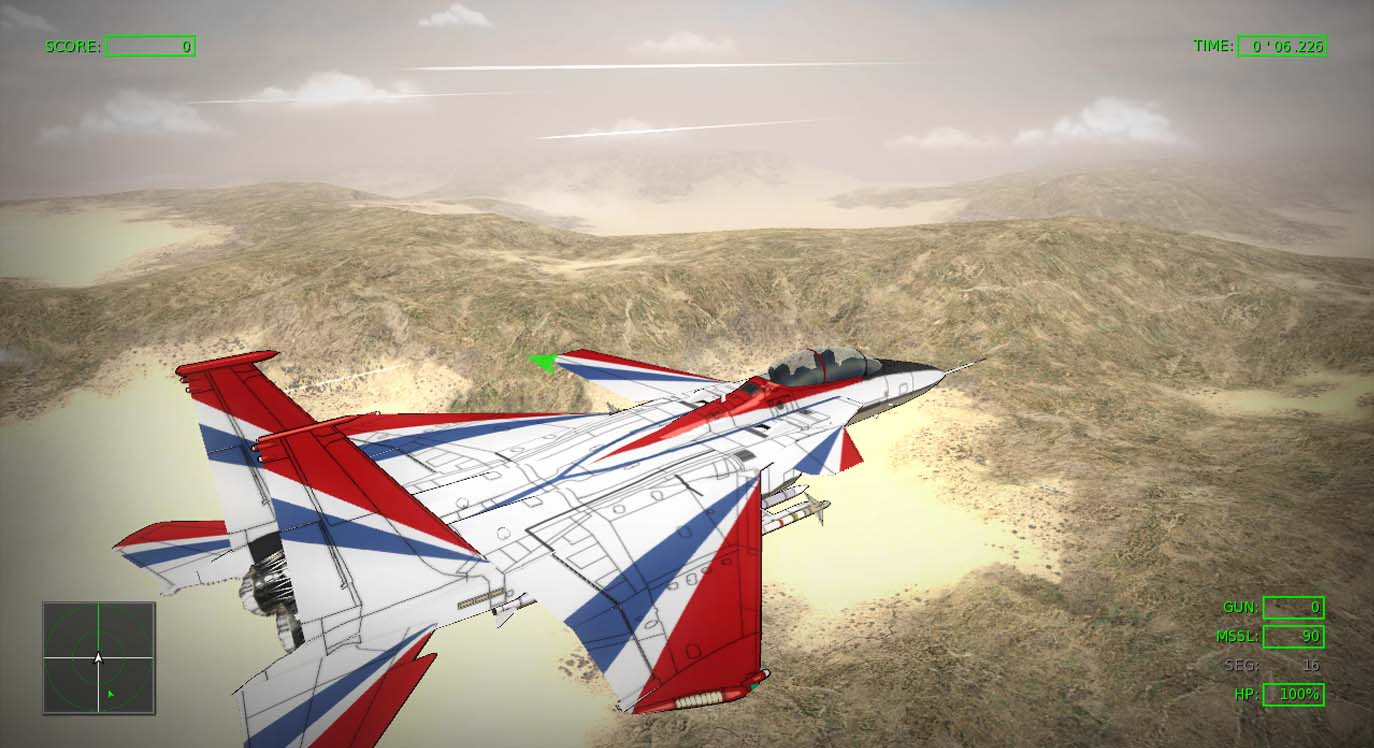And finally, pick a mission the change the map file and play.

like the last picture ... a lot of code :) but greattt if i have time need to study it

Still can't get my electrosphere map working might start fresh again.

is that the one with the tunnel you were showing on your project (which you should probably link on the main forums for publicity)?

Well, when you put it that way it sounds soooo easy! *Sarcasm self-test complete*.

Seriously, I'd get into doing the modding except I'm not terribly good at doing all of that complex stuff just quite so yet, which sucks as I've got lots of cool ideas running about (at least I think they're cool), and I have no idea how to use the SDKs and such.

In case you need, feel free to request a tutorial.

Also, if you are having trouble in apply your ideas to the game, we can discuss it in the forum or send me a private message and I’ll help in what you need.

Reply Good karma+1 vote

I request a tutorial for scripting missions, particularly what the numbers values you plug into the ___type variables do. Is there a list somewhere that I can look at?

Just start working on an asset and try to complete a little more of it every day, I guess.

So what's the best way to deal with really large buildings? Would you make, say, a dam part of the map geometry or place it as a separate object?

For a dam it works in both ways, but in case you what to make it destroyable is better as an object.

Reply Good karma+1 vote

I'm guessing that you have to place destructible objects via a text file, so what would be the best way to get the position of the breakable sections right?

what does wasWater do, and is the skybox/dome automatically created?

Actually… “_WasWater “ is wrong. That part it was copied from older data. As the map does not have any water I didn’t notice the issue.
The true command is:
Mat_#_HasWater = “bool”
(in the template on top it is right)

It adds a water layer just below the texture, but the texture must have at least some transparency to be able to see it.

About the sky box and sky dome they are not automatically created.
It requires adding a material file:
BGmaterial = "String"

(The Sky box can be very useful in creating space battles. )

Reply Good karma+1 vote

I only meant automatically created as in you don't have to model the geometry yourself, being able to use custom textures is always good.

That's a quick way to create basic geometry from a plane. I especially like the transition textures. In engines I used (CrySDK for example) the editor would deal with texture blending, while painting. Yet this here seems like a perfect way to do a very convincing version for the overall scene texture; I'll have to try it out.

How many transition texture pieces do you gather you used for the map? In theory it should be necessary to cover all angles, so at least 9 transition pieces? I really like these tuts!

Only registered members can share their thoughts. So come on! Join the community today (totally free - or sign in with your social account on the right) and join in the conversation.

Profile
Icon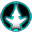Tutorial
Related Games